# R S Aggarwal Solutions for Class 10 Maths Chapter 17 Volume and Surface Area of Solids

Get RS Aggarwal Solutions for Class 10 Chapter 17 Volume and Surface Area of Solids here. We provide the solution for all exercise problems to help students in preparing for their exams. Class 10 Chapter 17 is based on volume and surface area of various solids such as a cube, cuboid, cylinder, sphere and their combinations. This is one of the important and interesting concepts in class 10 syllabus. The subject experts at BYJU’S have devised detailed solutions for the students to understand the concepts easily. Students can downloadR S Aggarwal Maths Class 10 Solutions now for free and start practising.

## Download PDF of R S Aggarwal Solutions for Class 10 Chapter 17 Volume and Surface Area of Solids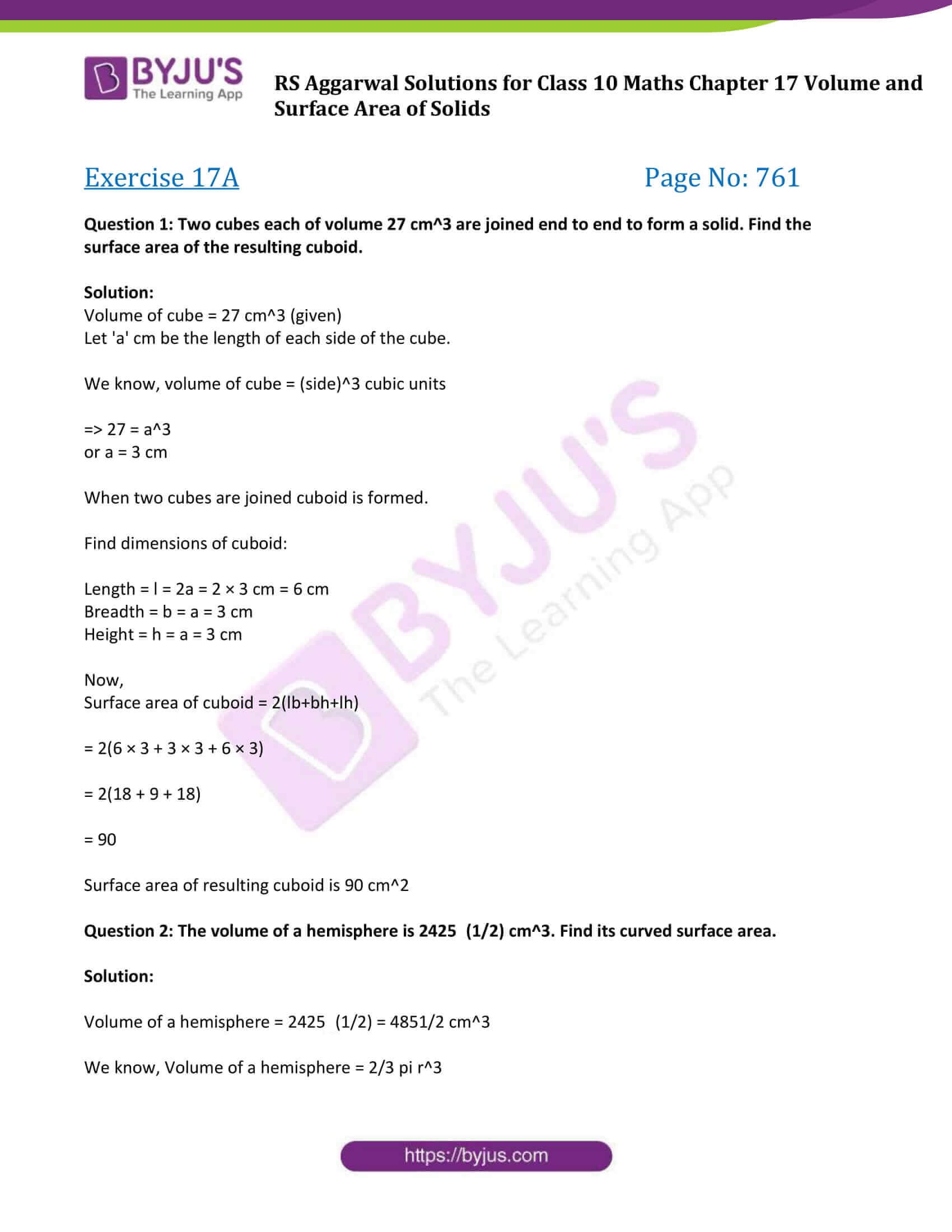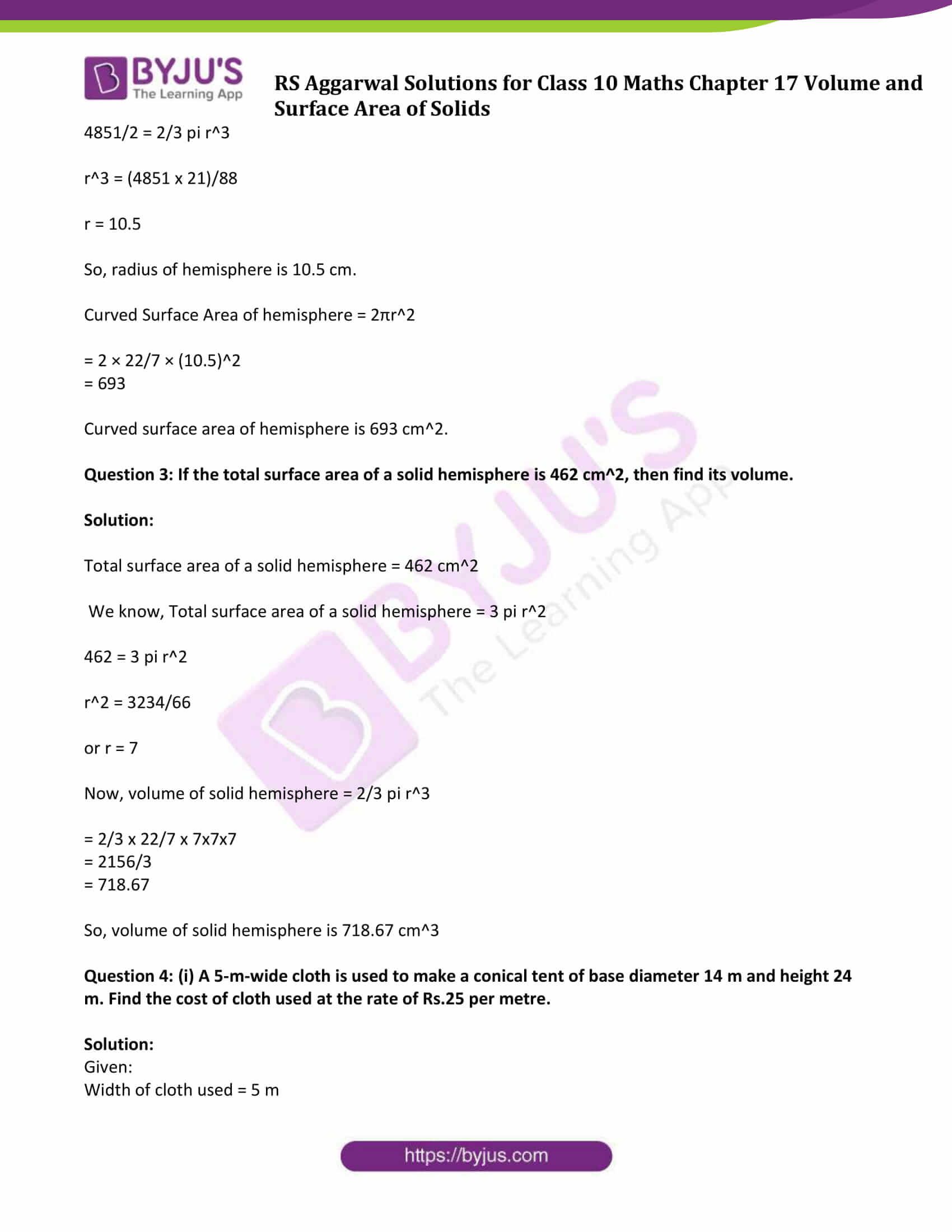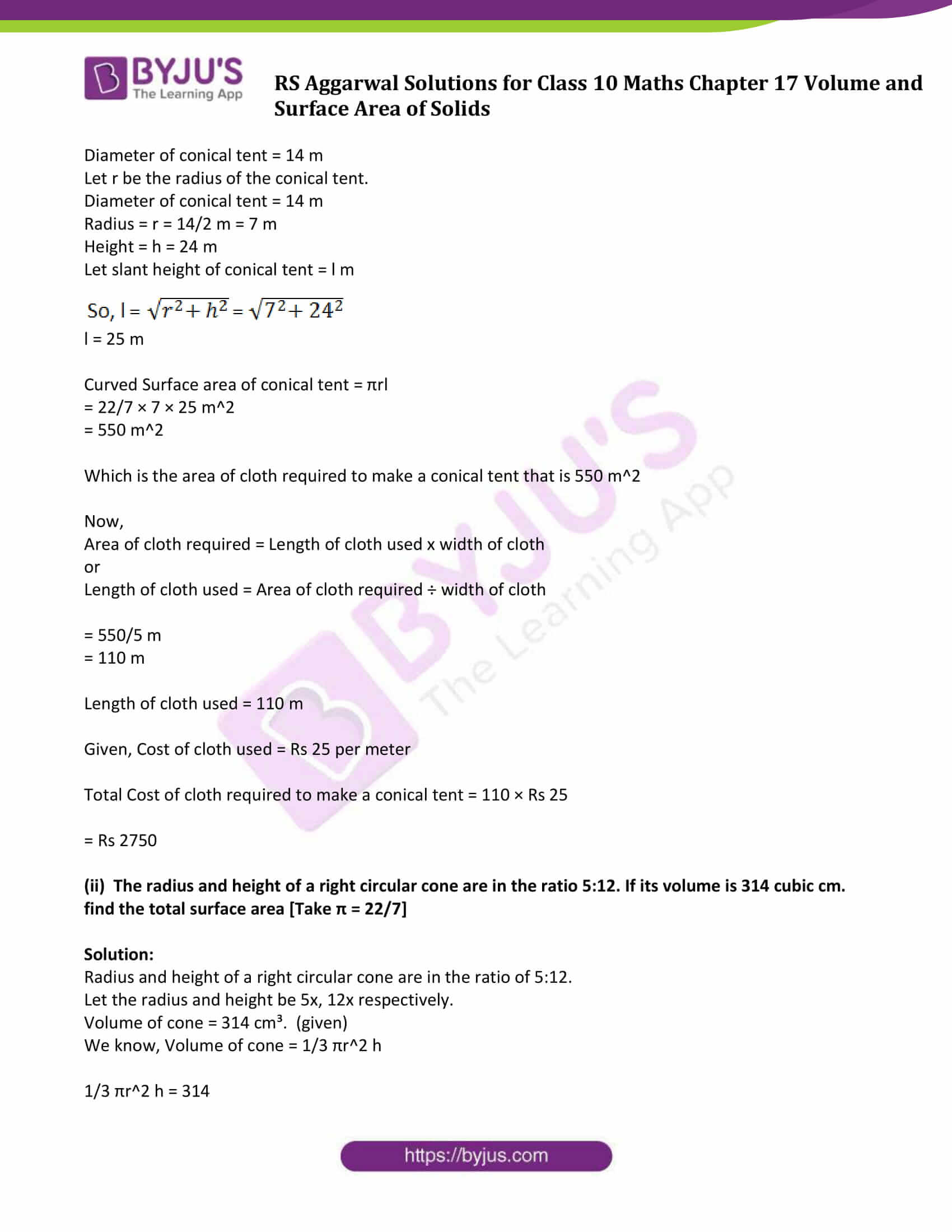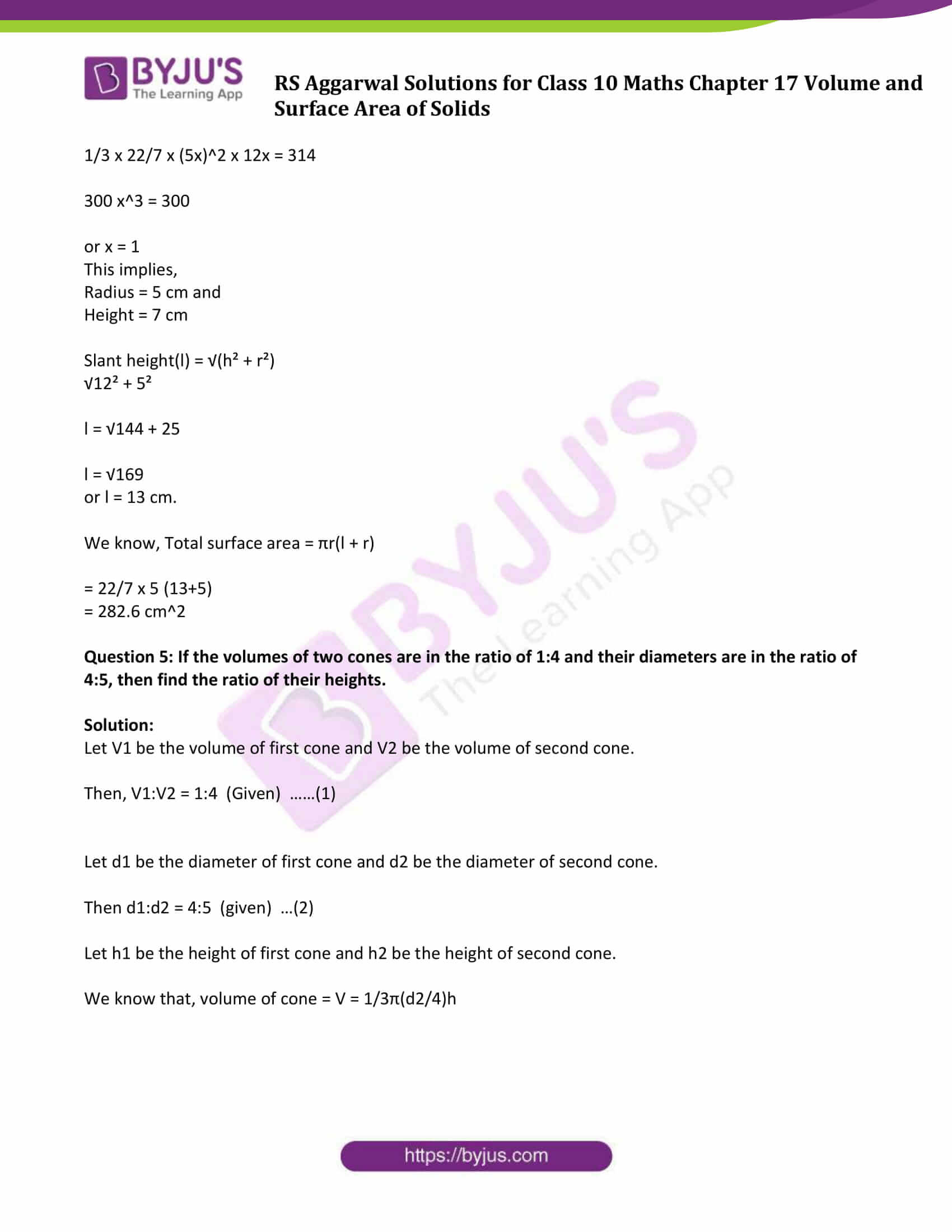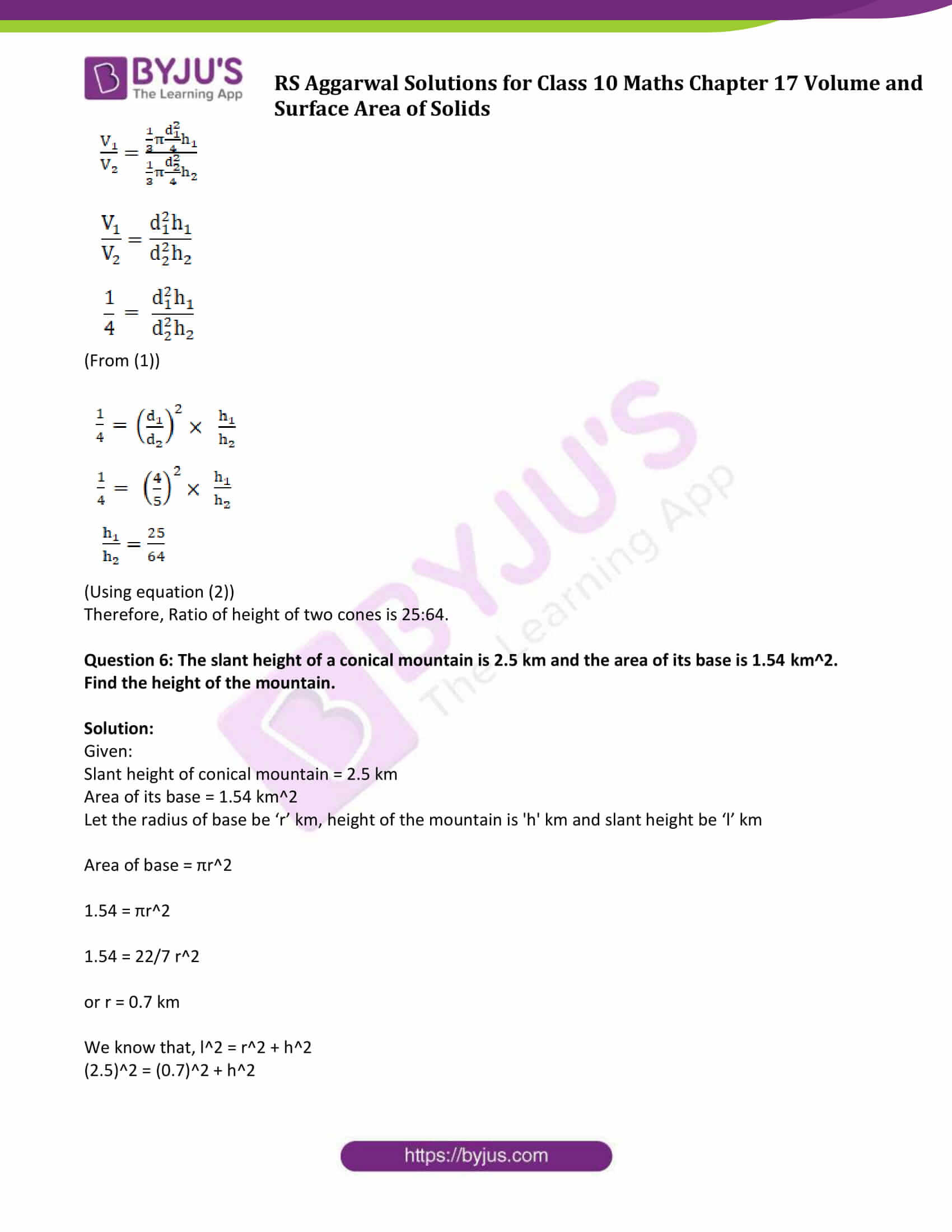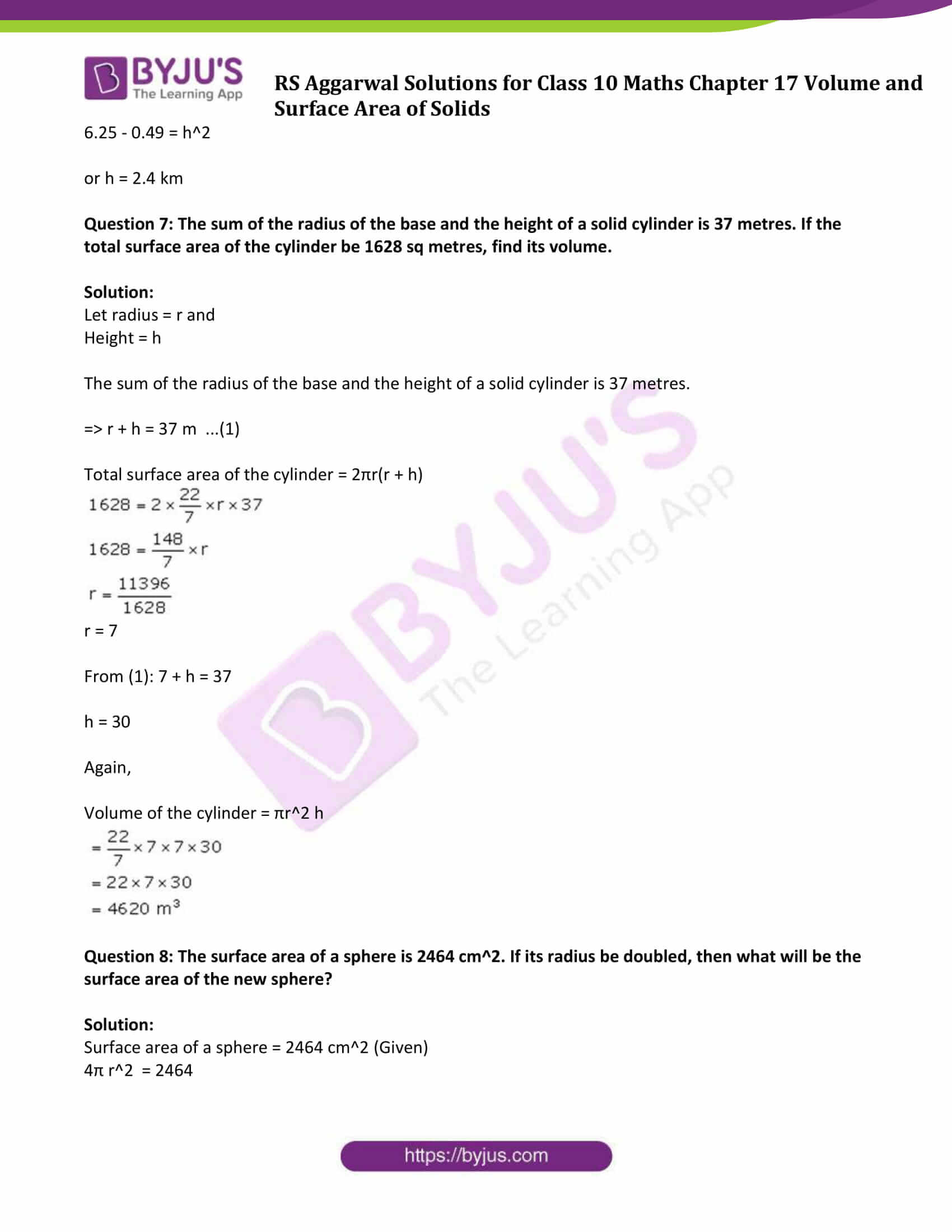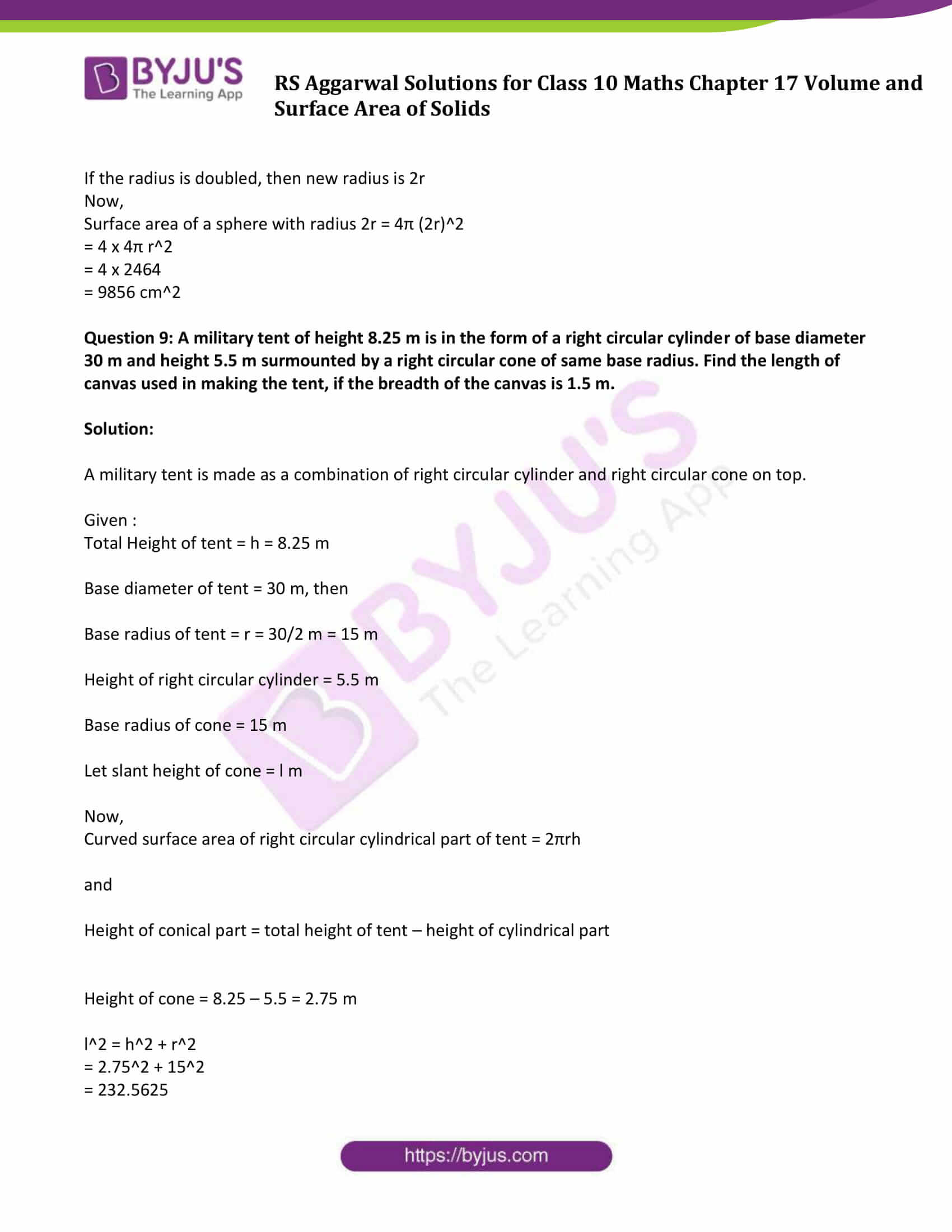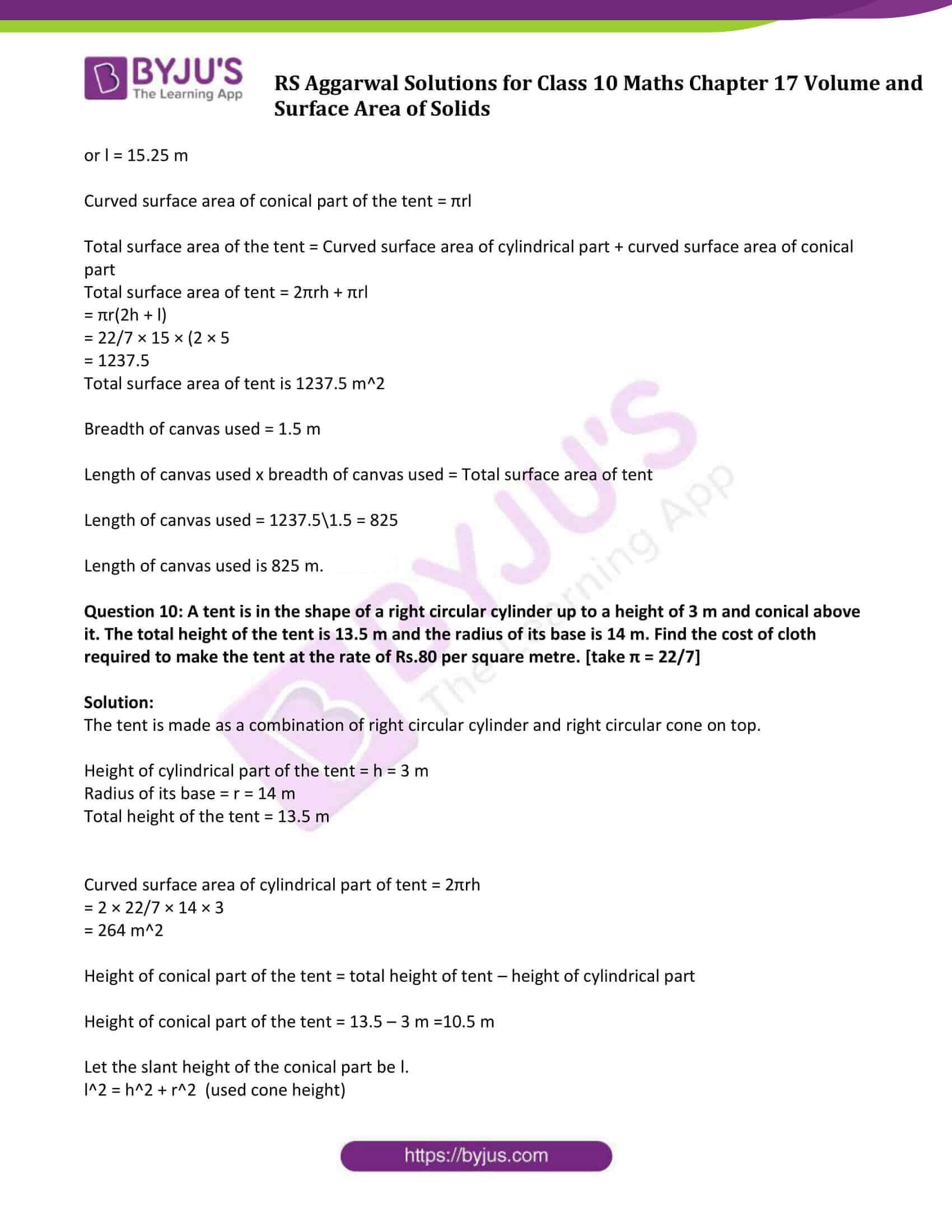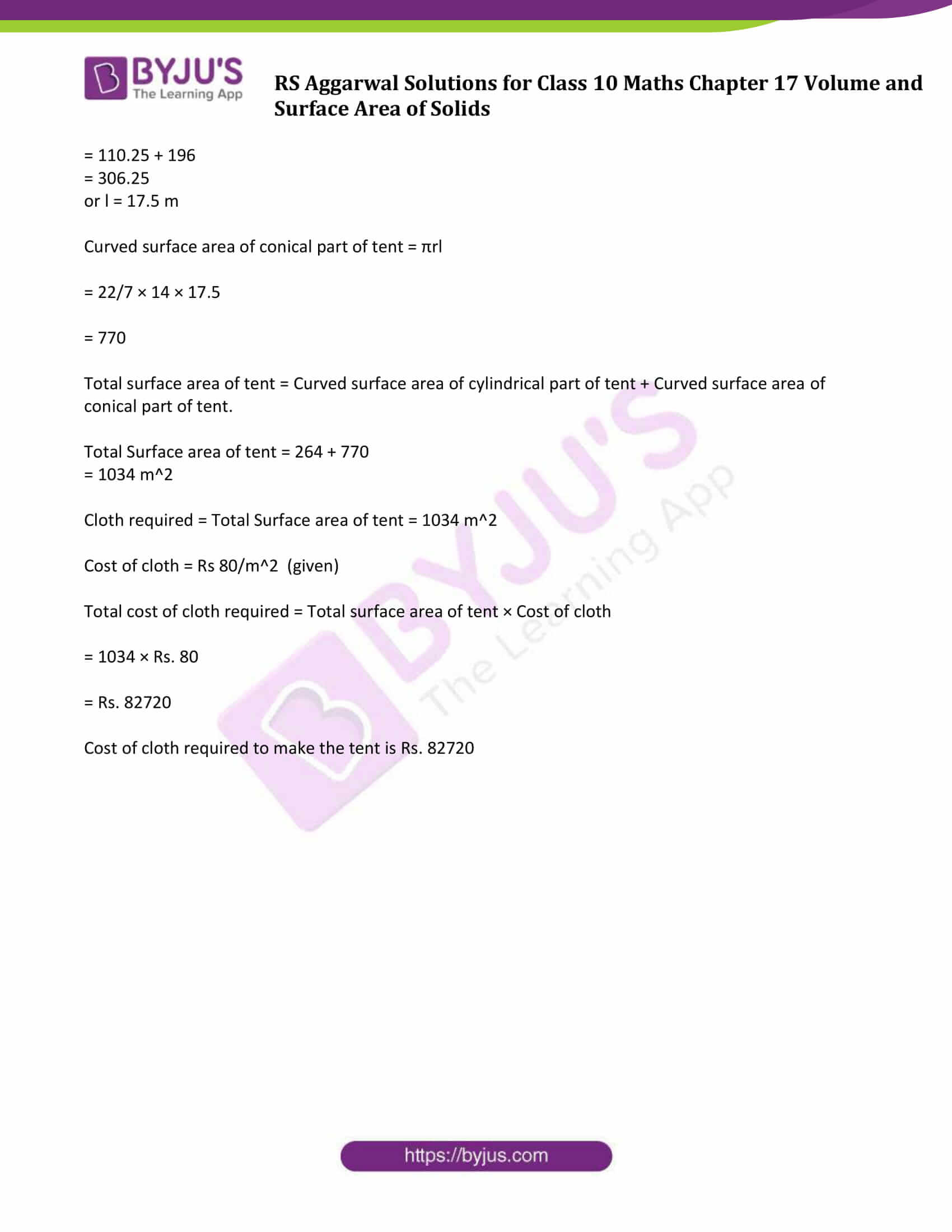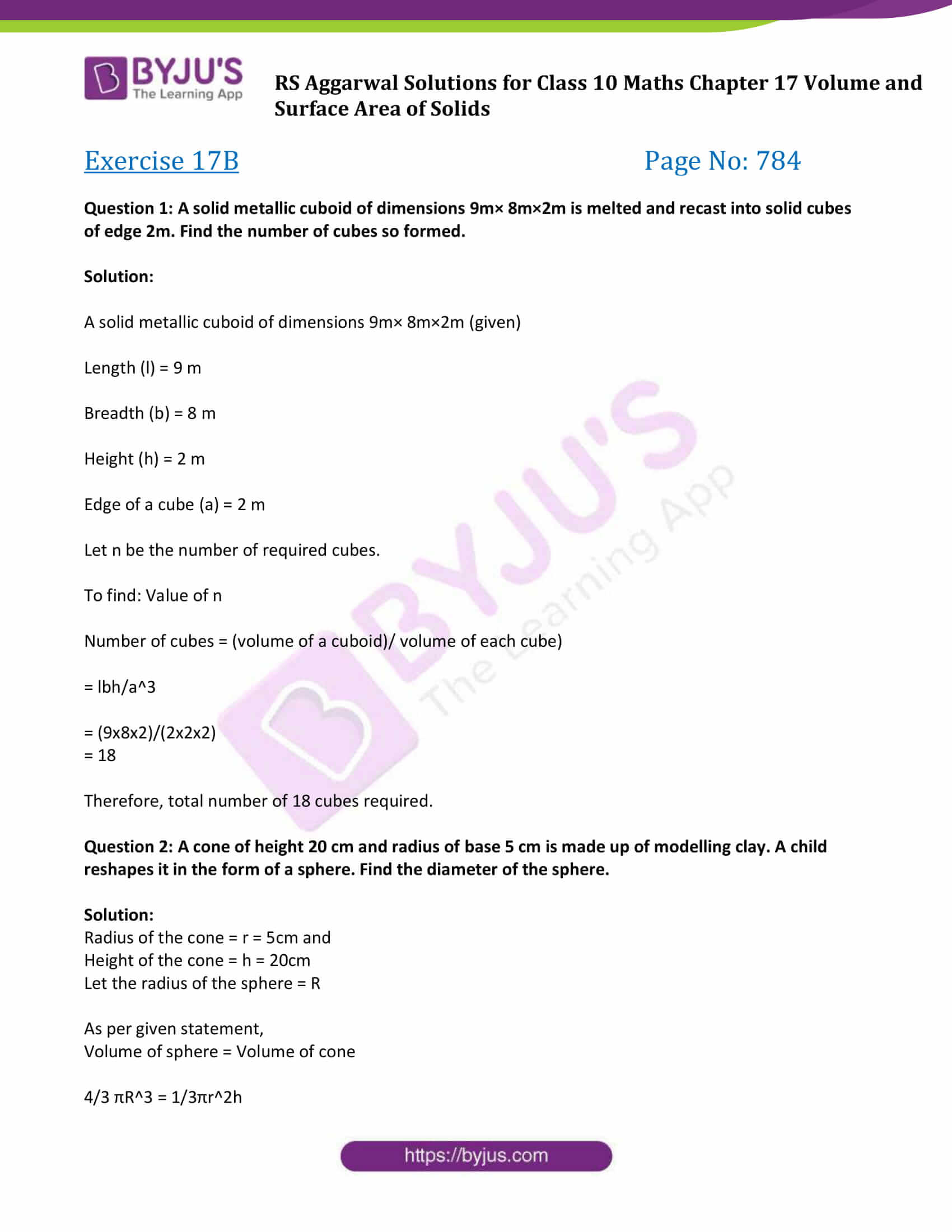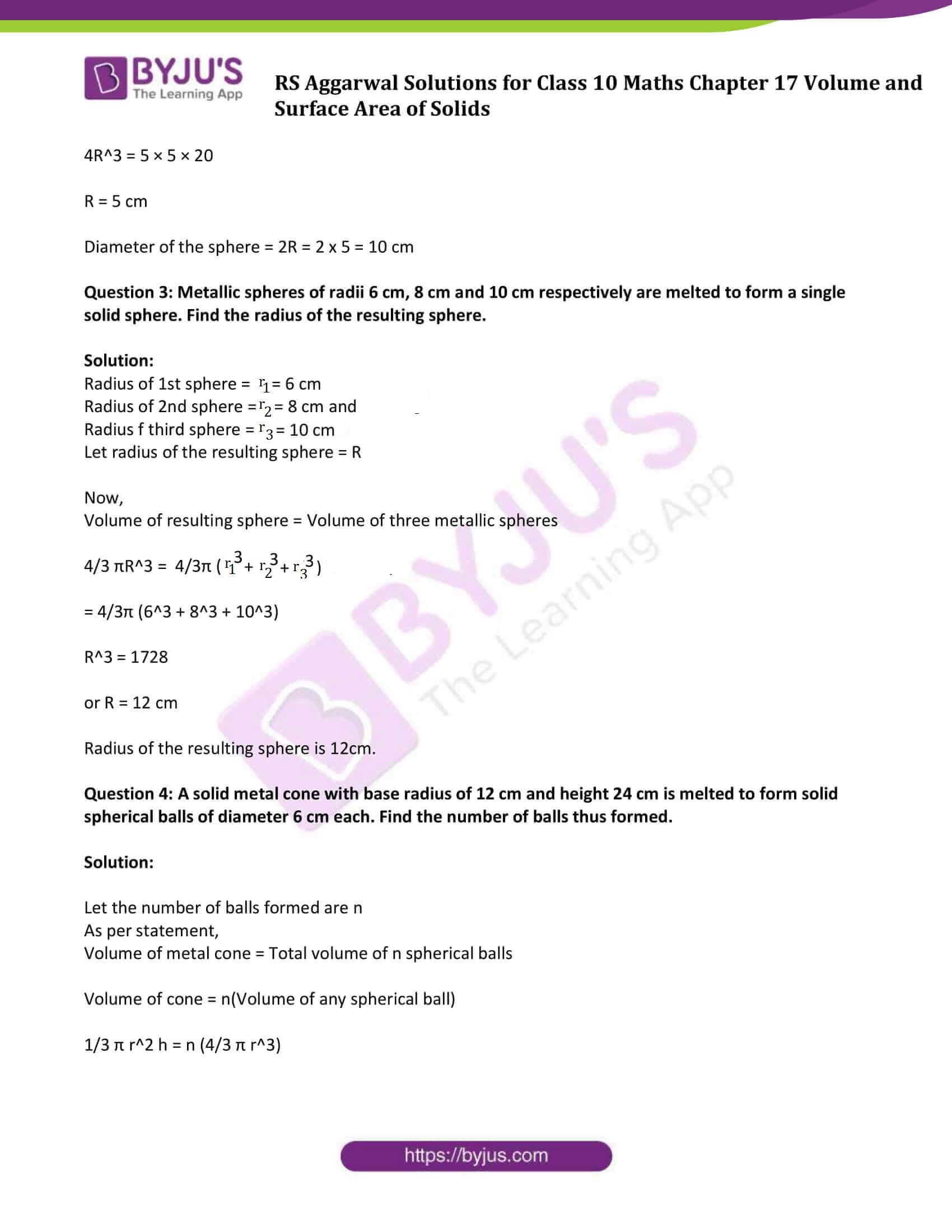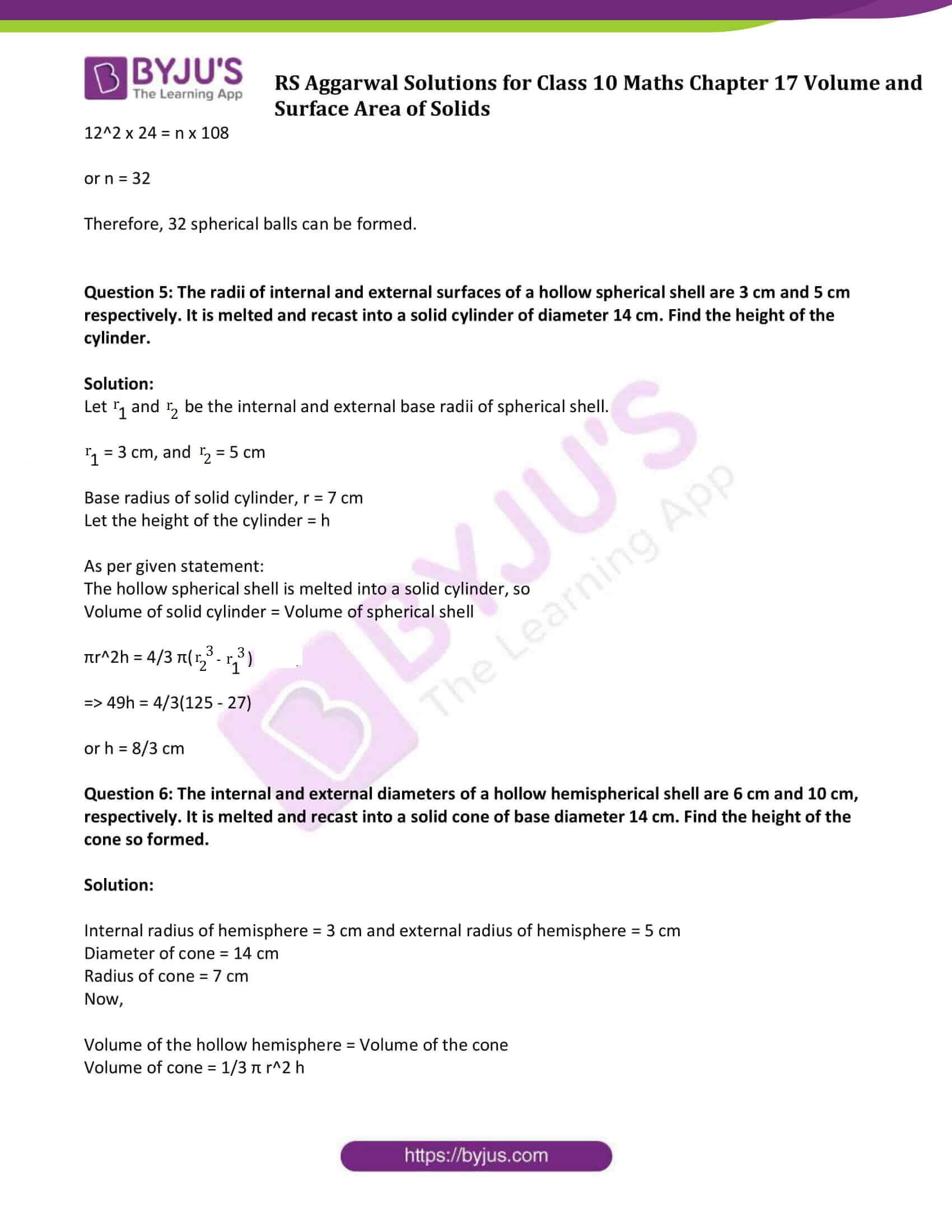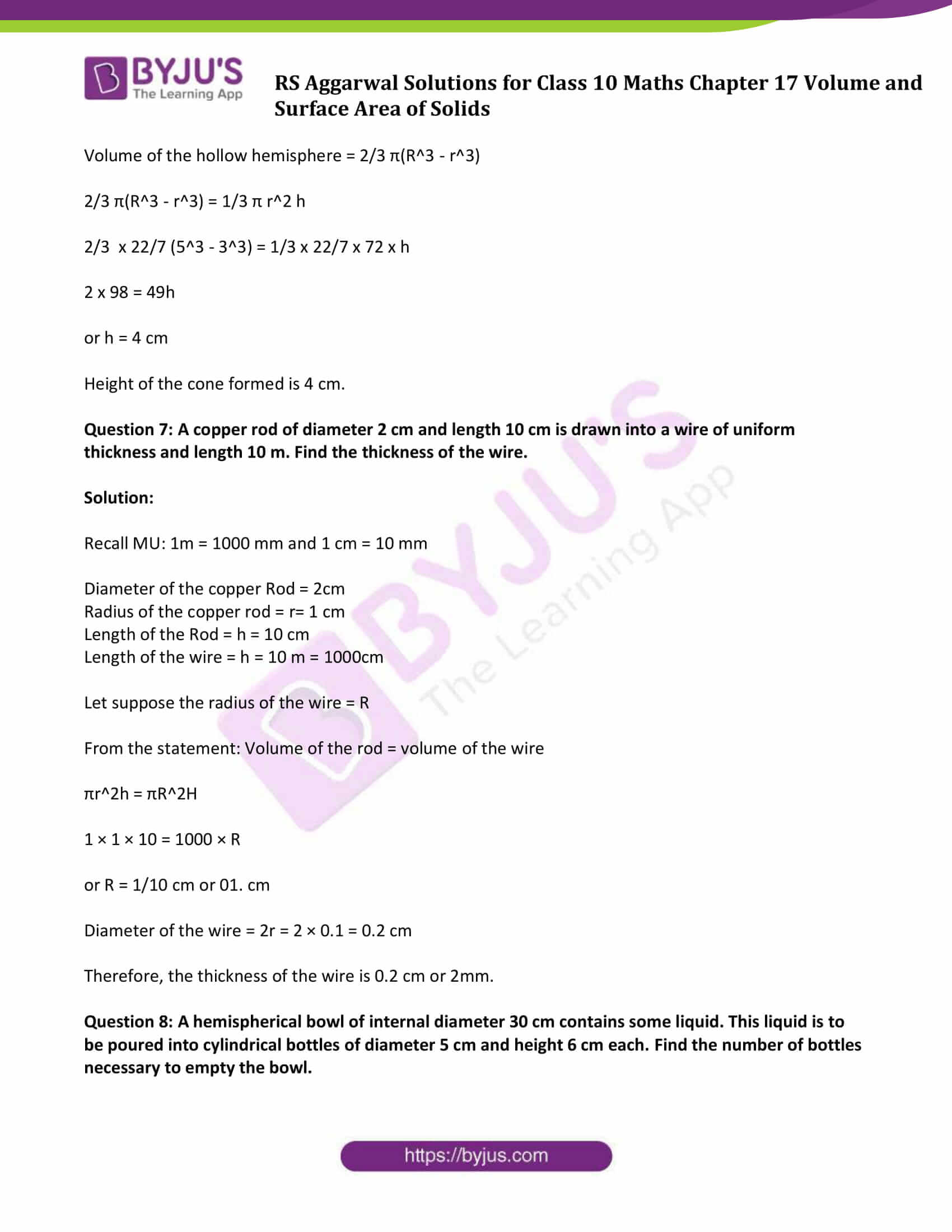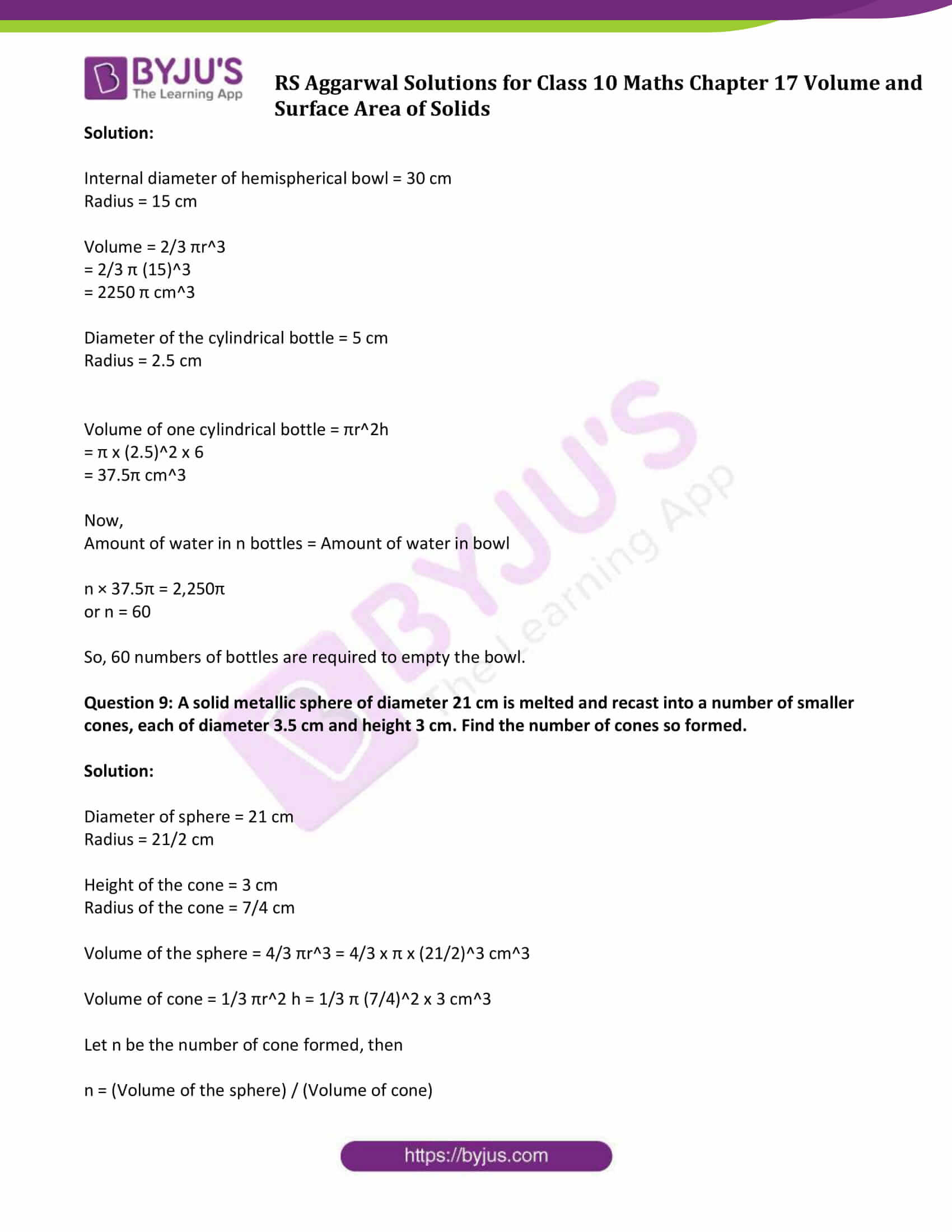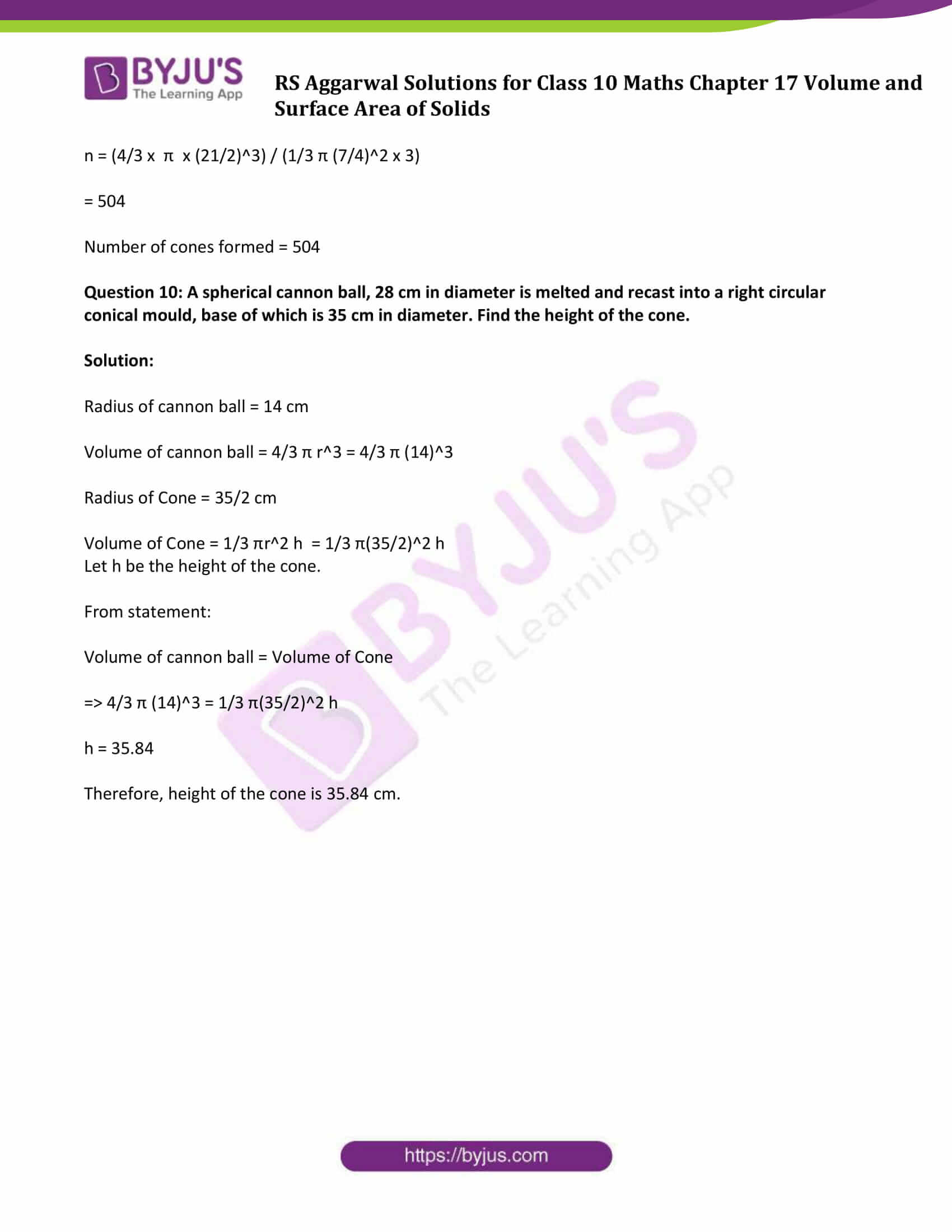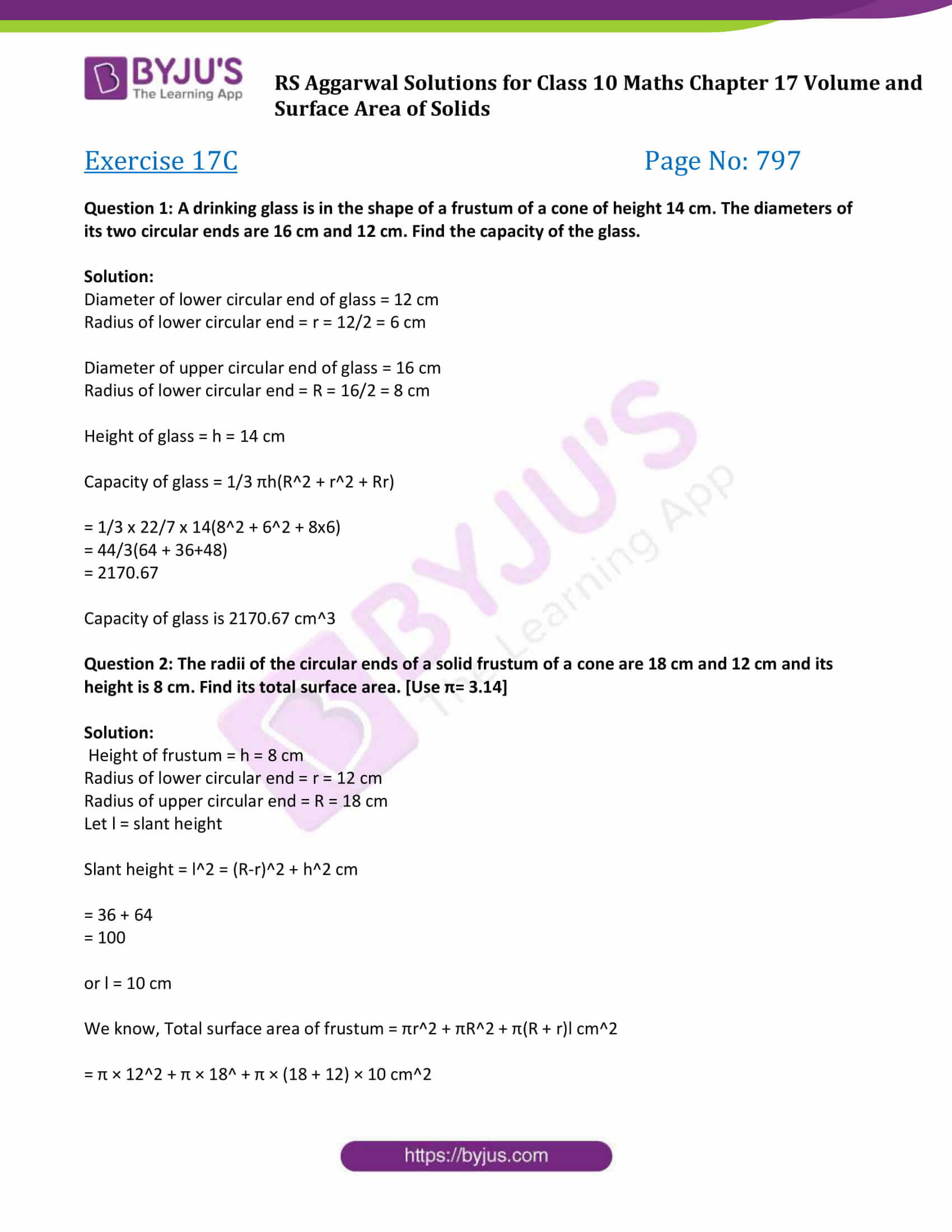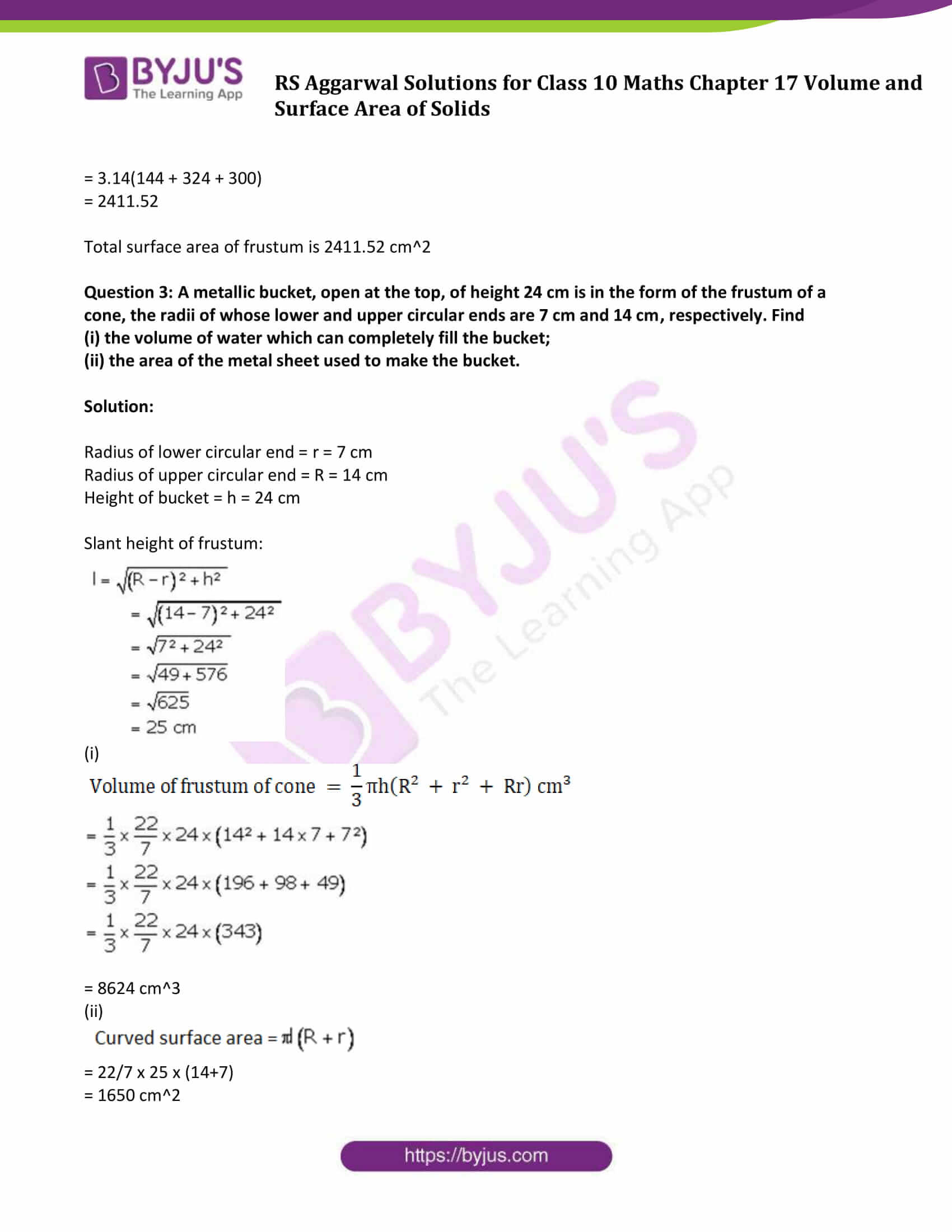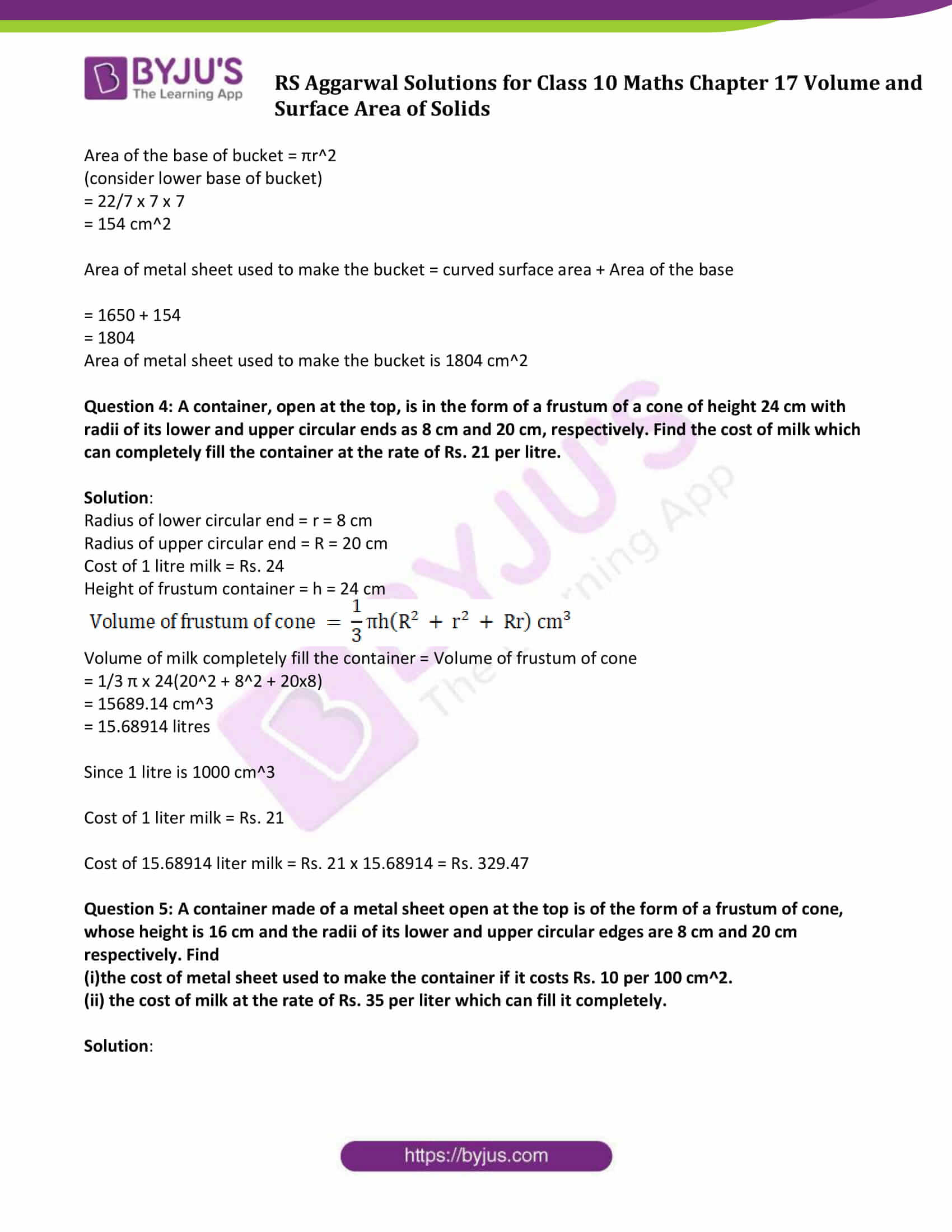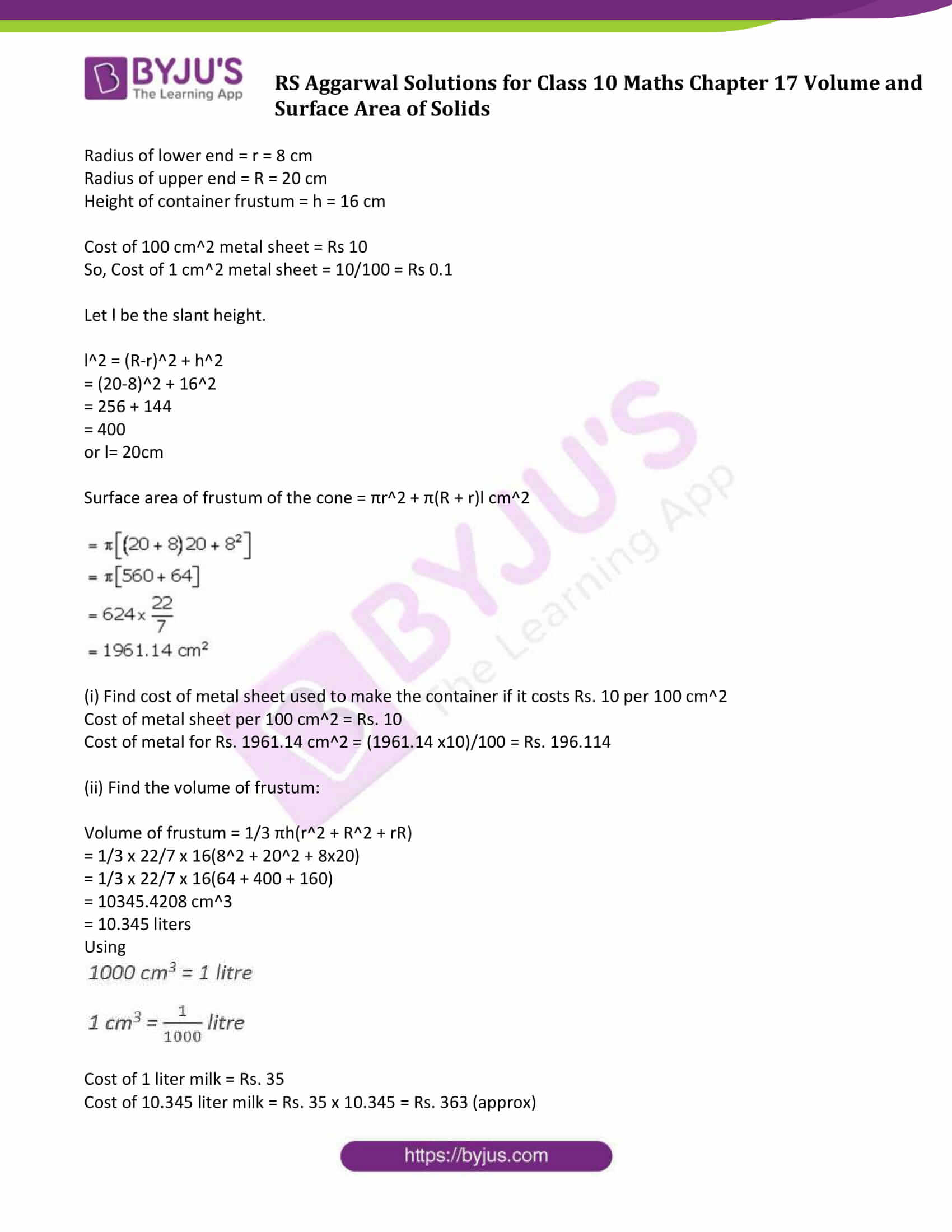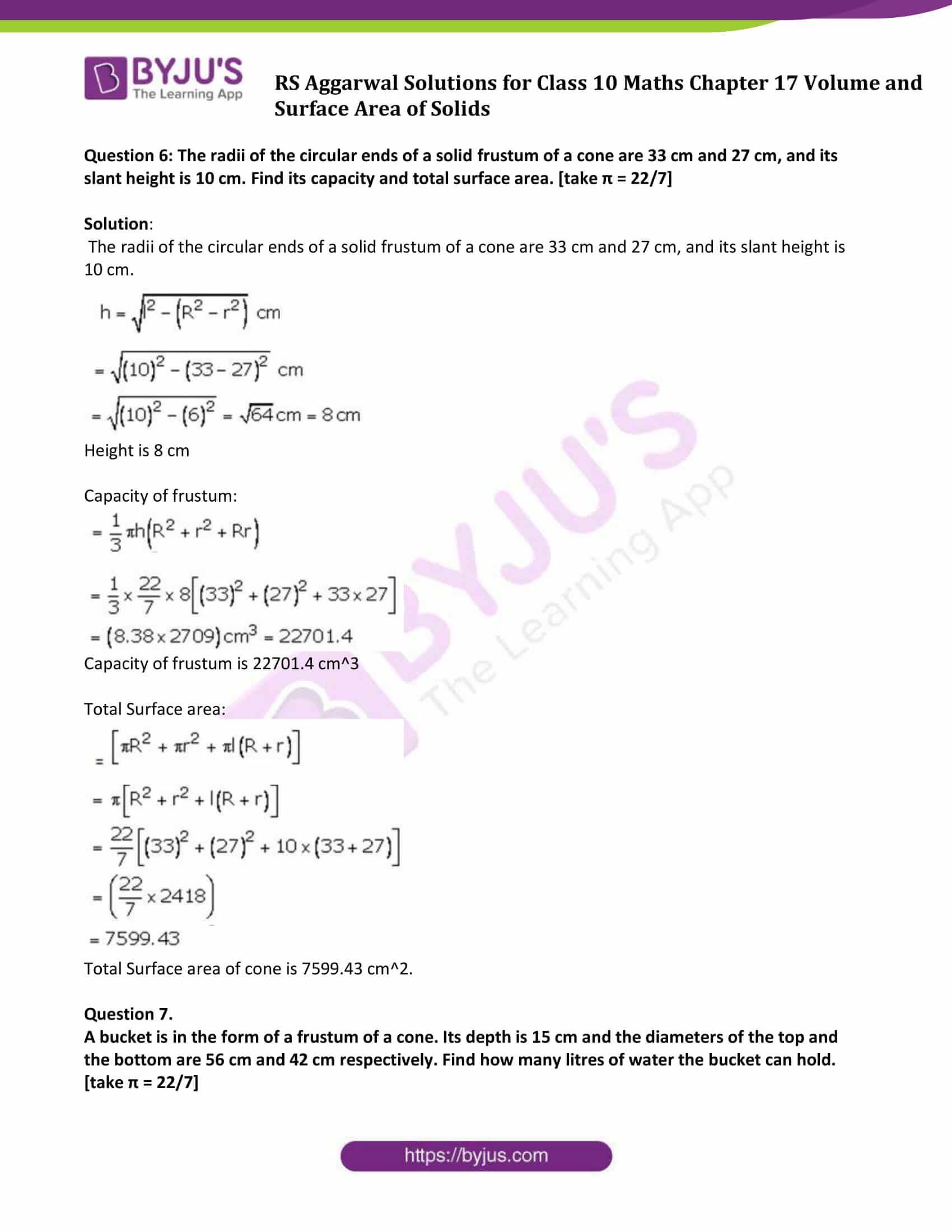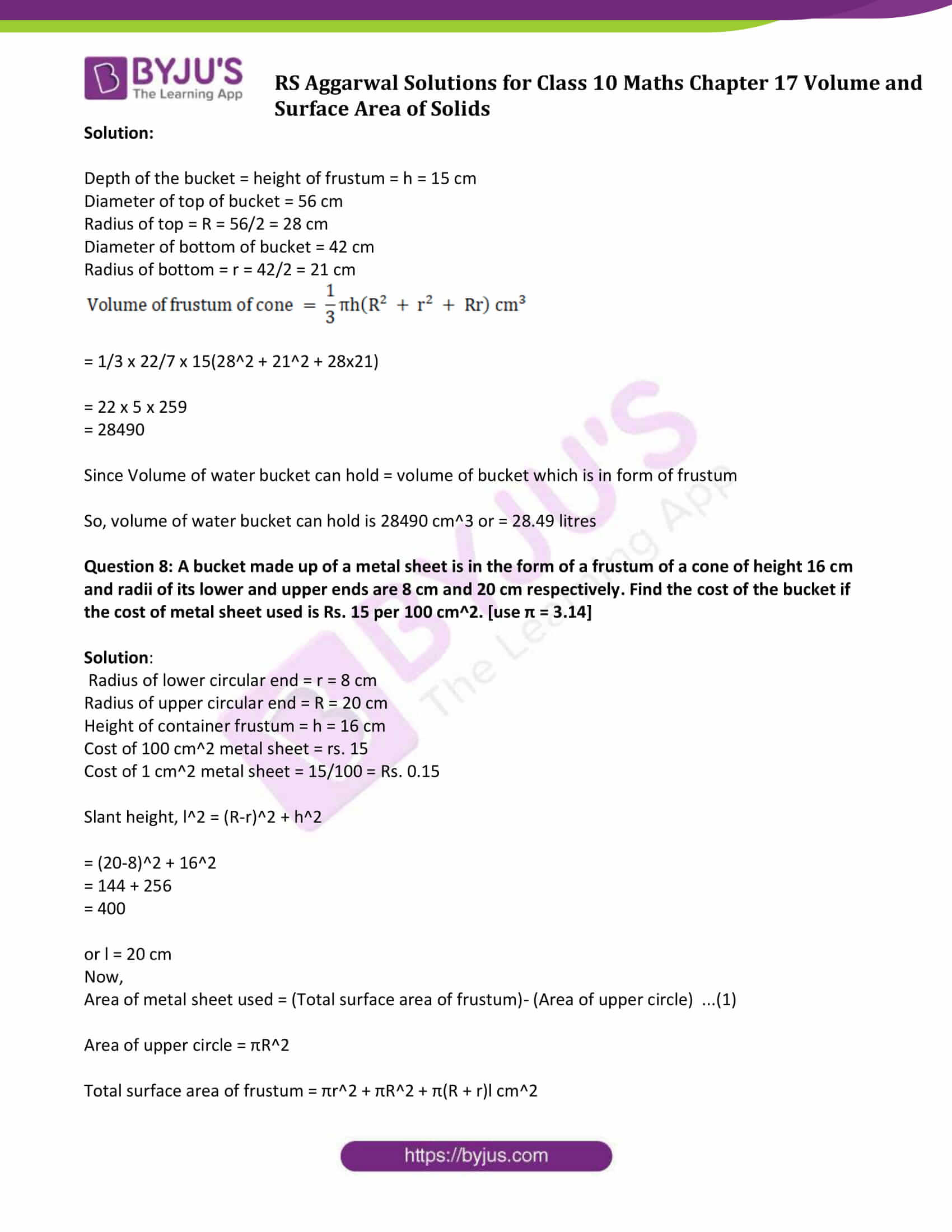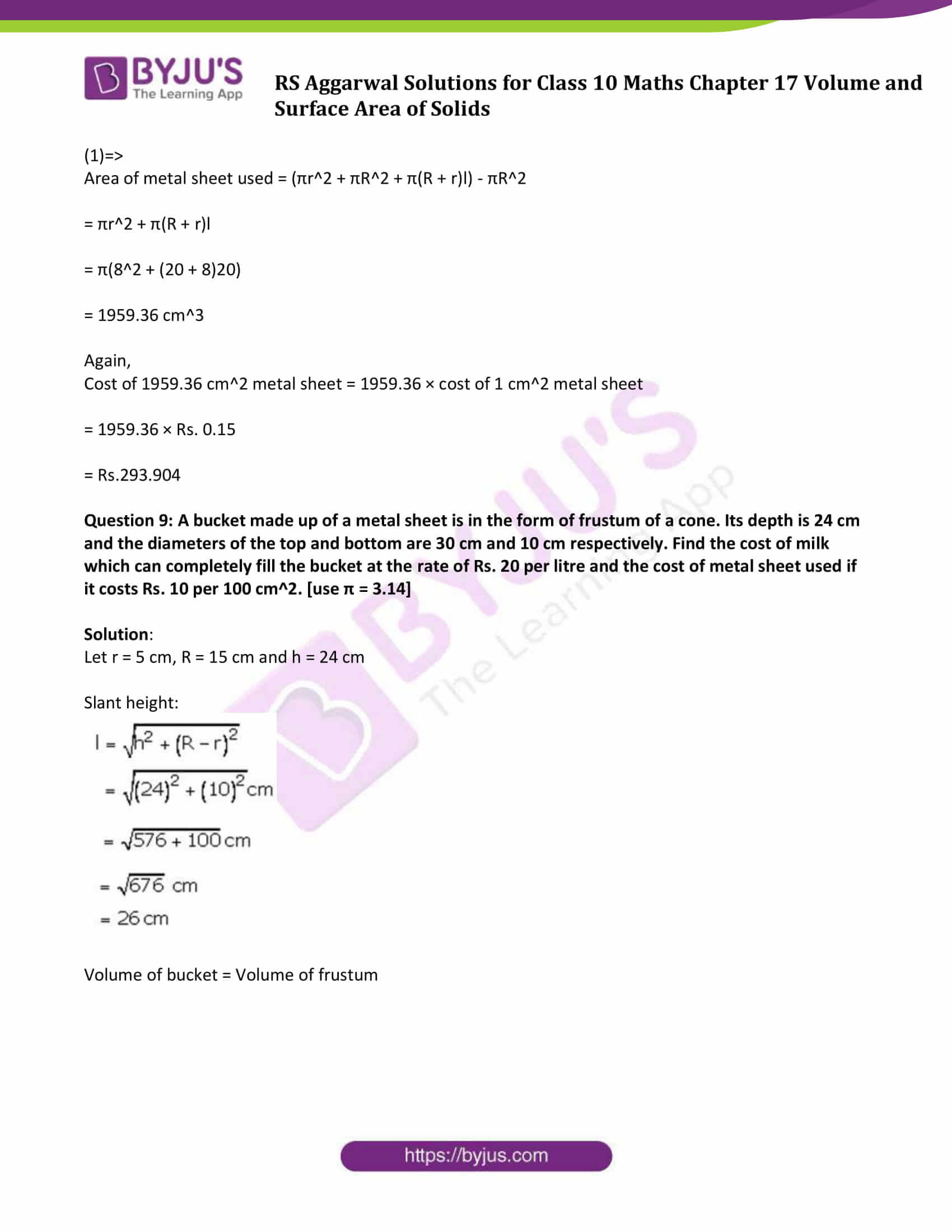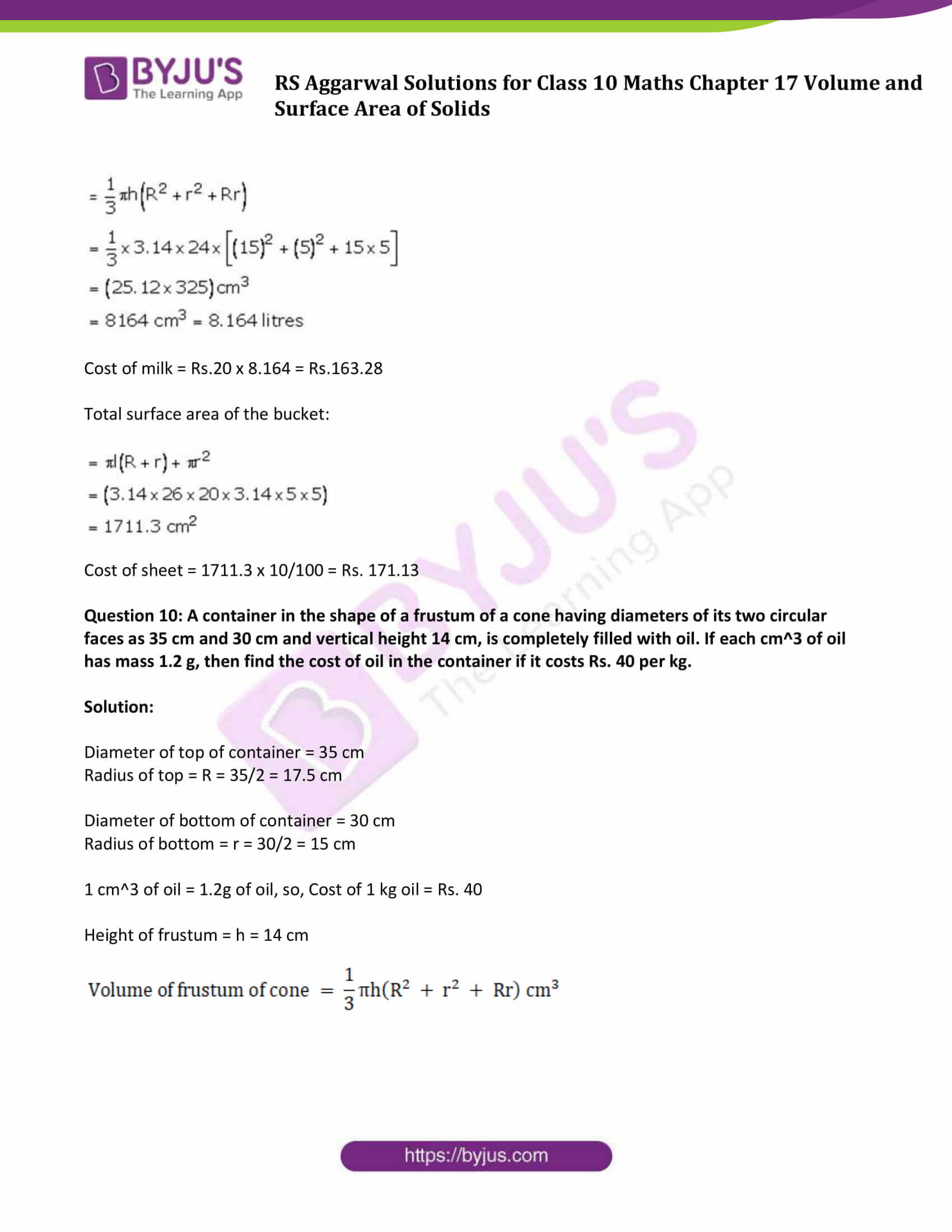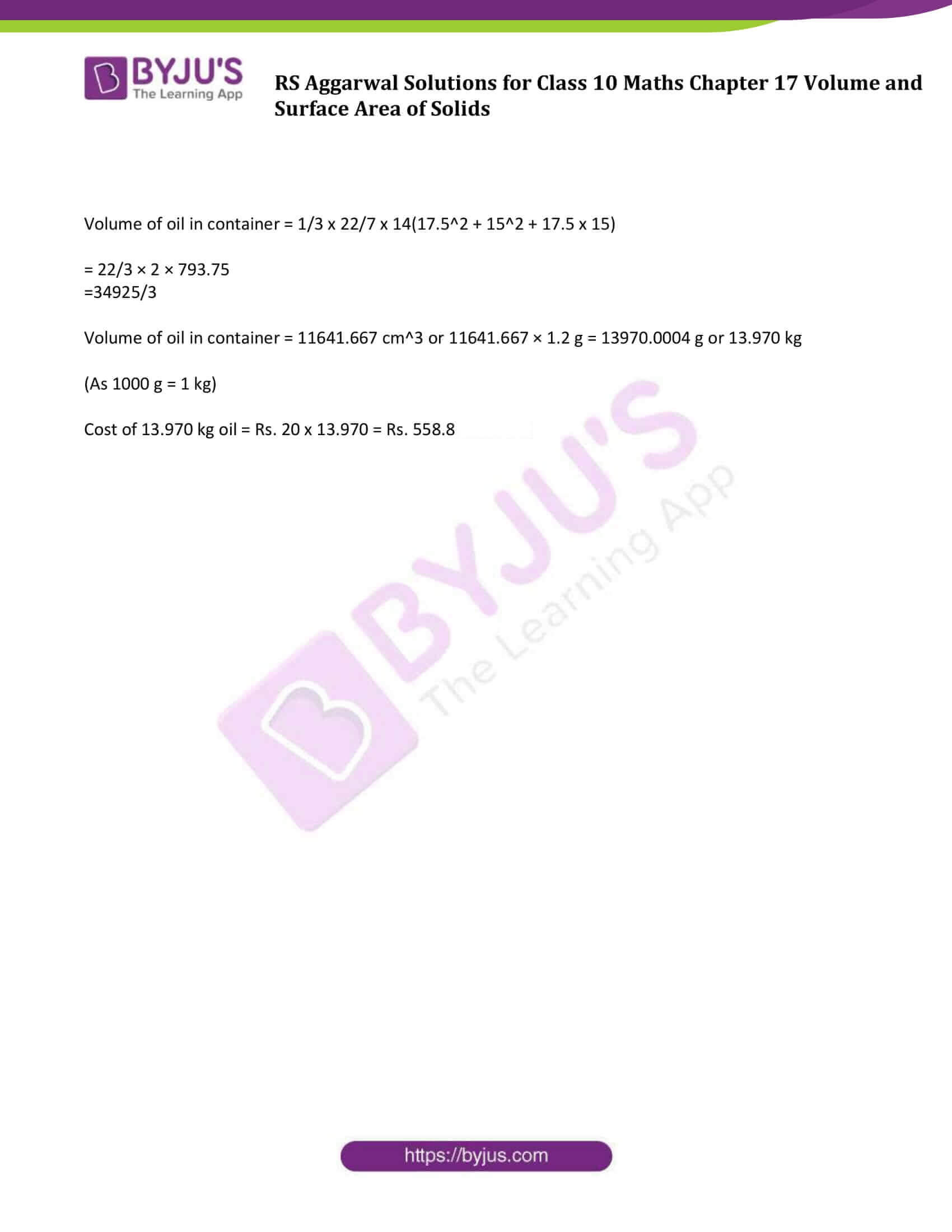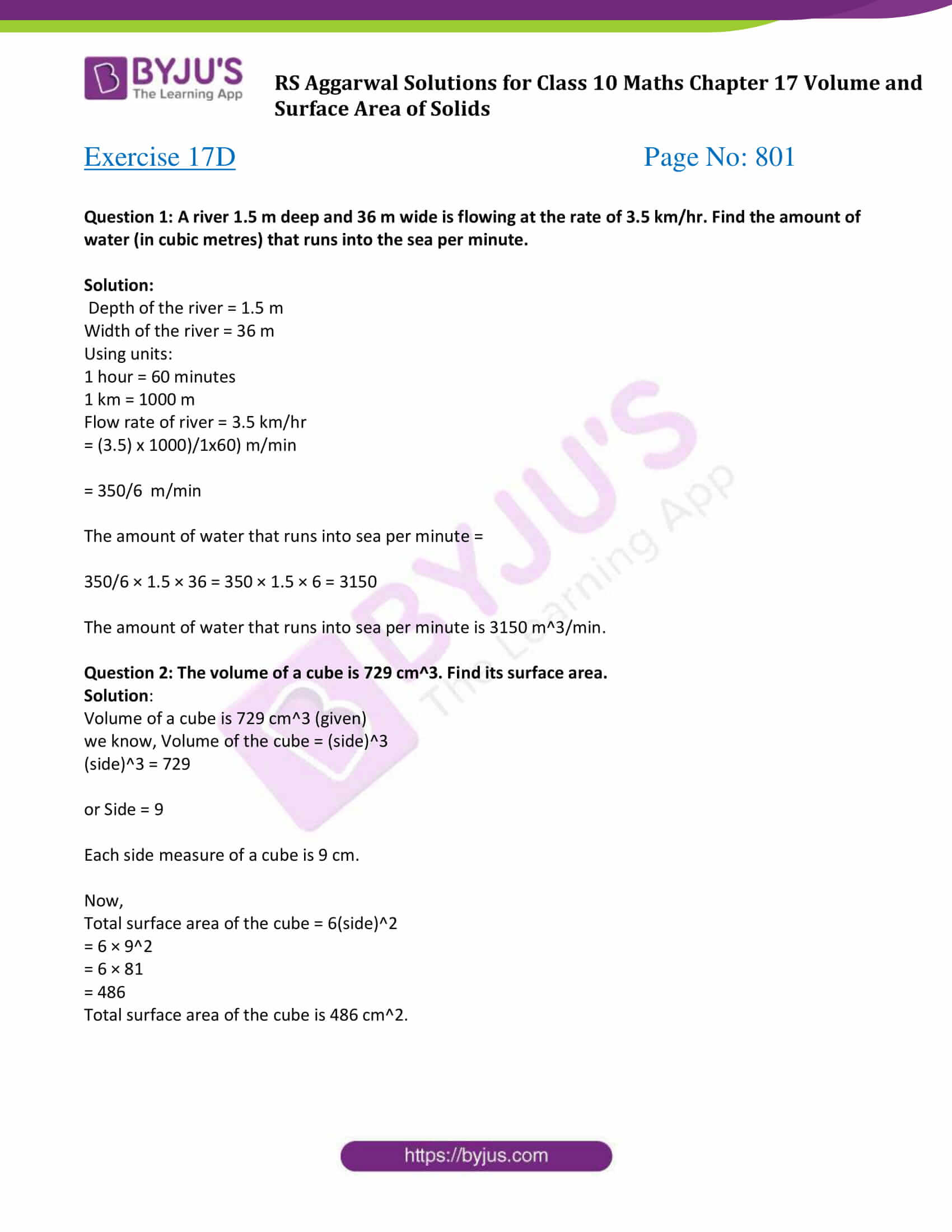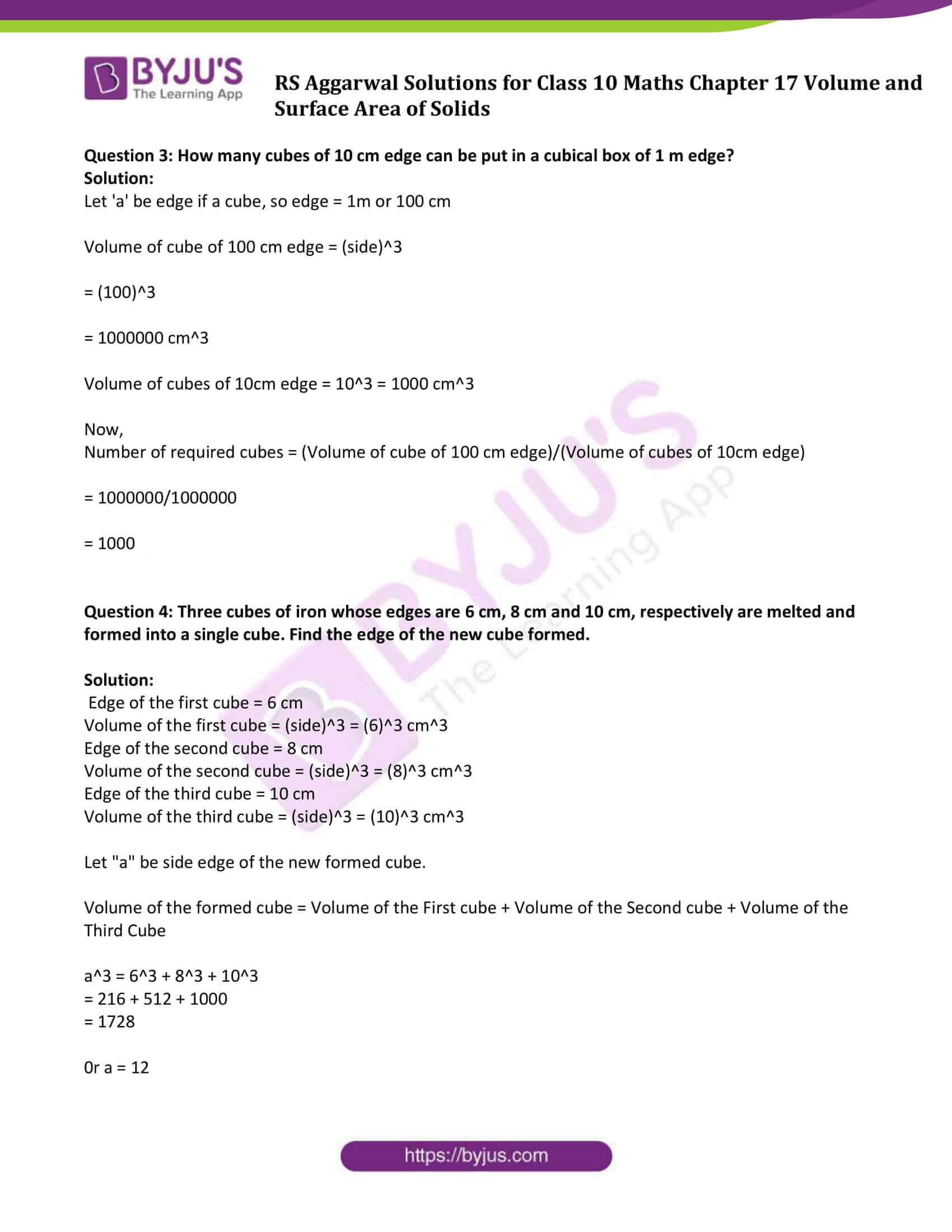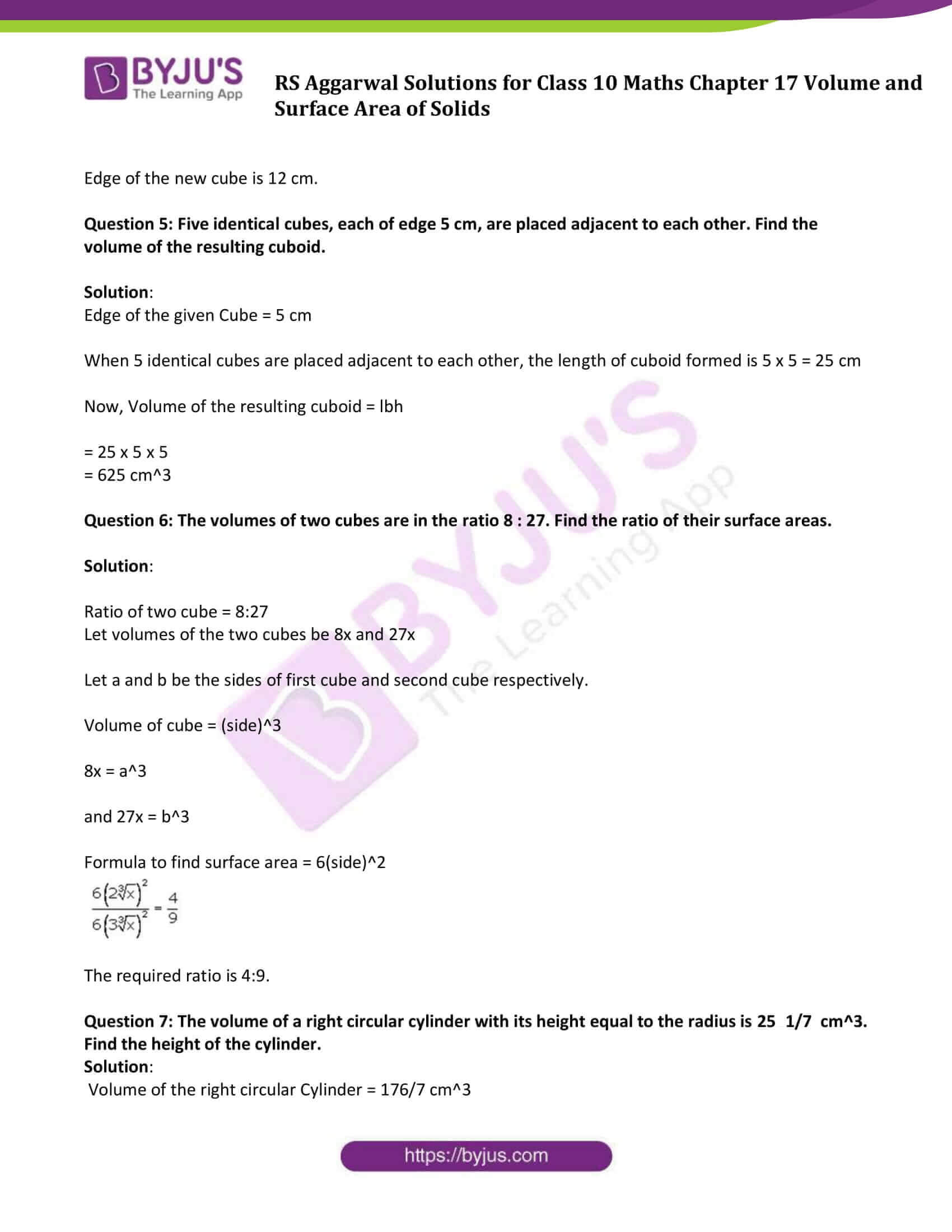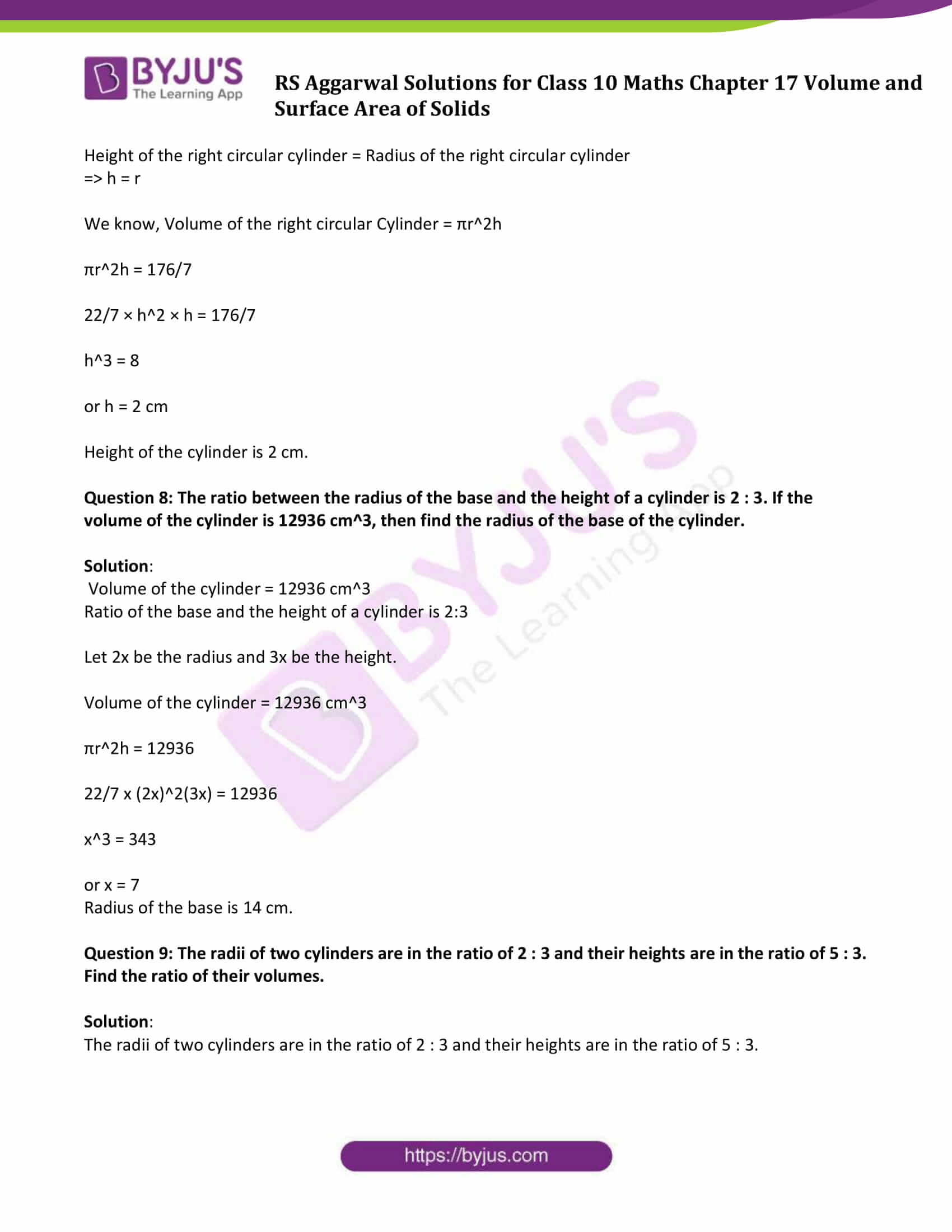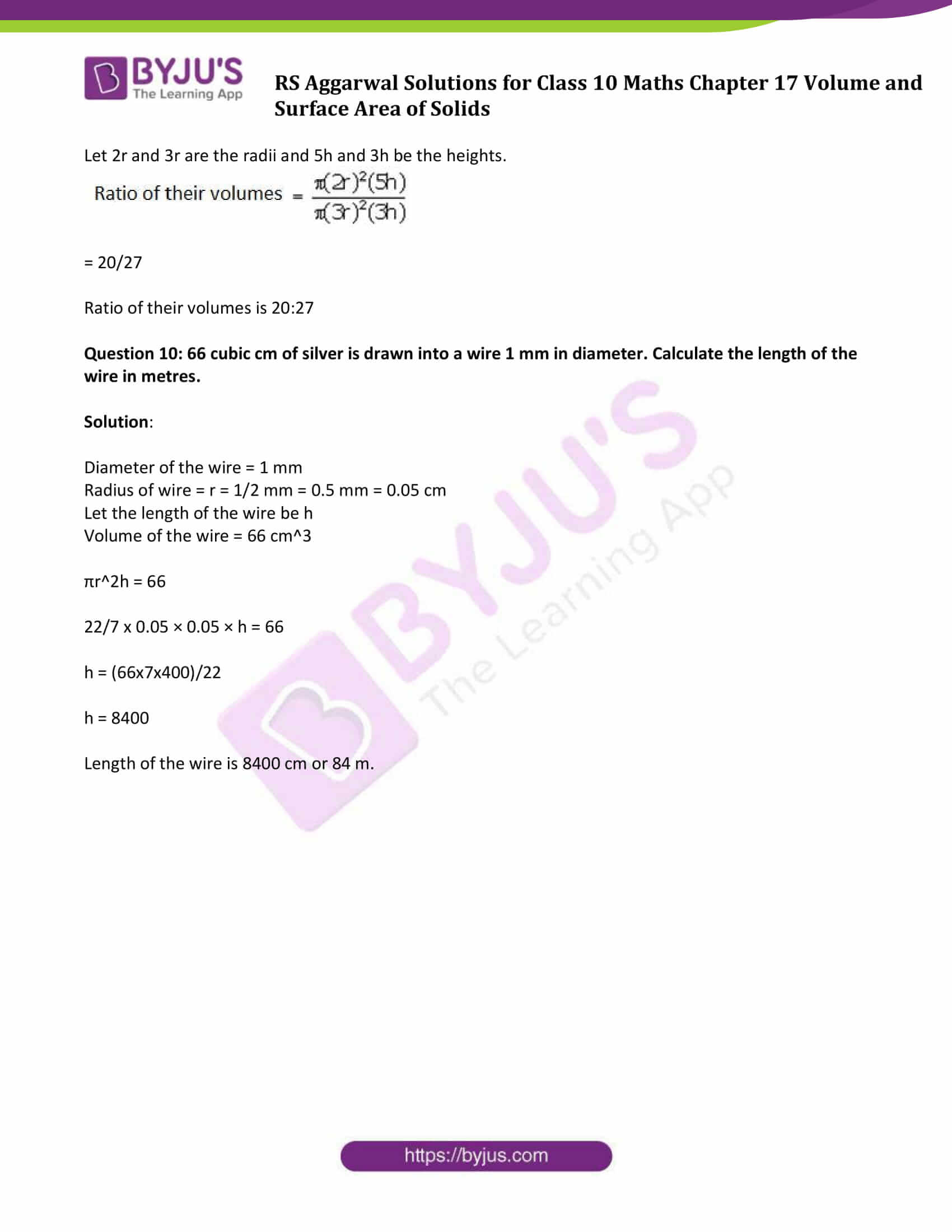### Access Solutions of Maths R S Aggarwal Chapter 17 – Volume and Surface Area of Solids

Get detailed solutions for all the questions listed under the below exercises:

Exercise 17 A Solutions

Exercise 17 B Solutions

Exercise 17 C Solutions

Exercise 17 D Solutions

## Exercise 17A

Question 1: Two cubes each of volume 27 cm3 are joined end to end to form a solid. Find the surface area of the resulting cuboid.

Solution:

Volume of cube = 27 cm3 (given)

Let ‘a’ cm be the length of each side of the cube.

We know, volume of cube = (side)3 cubic units

⇨ 27 = a3

or a = 3 cm

When two cubes are joined cuboid is formed.

Find dimensions of cuboid:

Length = l = 2a = 2 × 3 cm = 6 cm

Breadth = b = a = 3 cm

Height = h = a = 3 cm

Now,

Surface area of cuboid = 2(lb+bh+lh)

= 2(6 × 3 + 3 × 3 + 6 × 3)

= 2(18 + 9 + 18)

= 90

Surface area of resulting cuboid is 90 cm2

Question 2: The volume of a hemisphere is 2425(1/2) cm3. Find its curved surface area.

Solution:

Volume of a hemisphere = 2425(1/2) = 4851/2 cm3

We know, Volume of a hemisphere = 2/3 pi r3

4851/2 = 2/3 pi r3

r3 = (4851 x 21)/88

r = 10.5

So, radius of hemisphere is 10.5 cm.

Curved Surface Area of hemisphere = 2πr2

= 2 × 22/7 × (10.5)2

= 693

Curved surface area of hemisphere is 693 cm2.

Question 3: If the total surface area of a solid hemisphere is 462 cm2, then find its volume.

Solution:

Total surface area of a solid hemisphere = 462 cm2

We know, Total surface area of a solid hemisphere = 3 pi r2

462 = 3 pi r2

r2 = 3234/66

or r = 7

Now, volume of solid hemisphere = 2/3 pi r3

= 2/3 x 22/7 x 7x7x7

= 2156/3

= 718.67

So, volume of solid hemisphere is 718.67 cm3

Question 4: (i) A 5-m-wide cloth is used to make a conical tent of base diameter 14 m and height 24 m. Find the cost of cloth used at the rate of Rs.25 per metre.

Solution:

Given:

Width of cloth used = 5 m

Diameter of conical tent = 14 m

Let r be the radius of the conical tent.

Diameter of conical tent = 14 m

Radius = r = 14/2 m = 7 m

Height = h = 24 m

Let slant height of conical tent = l m

So , $I = \sqrt{r^{2}+h^{2}} \,=\, \sqrt{7^{2}+24^{2}}$

l = 25 m

Curved Surface area of conical tent = πrl

= 22/7 × 7 × 25 m2

= 550 m2

Which is the area of cloth required to make a conical tent that is 550 m2

Now,

Area of cloth required = Length of cloth used x width of cloth

or

Length of cloth used = Area of cloth required ÷ width of cloth

= 550/5 m

= 110 m

Length of cloth used = 110 m

Given, Cost of cloth used = Rs 25 per meter

Total Cost of cloth required to make a conical tent = 110 × Rs 25

= Rs 2750

(ii) The radius and height of a right circular cone are in the ratio 5:12. If its volume is 314 cubic cm. find the total surface area [Take π = 22/7]

Solution:

Radius and height of a right circular cone are in the ratio of 5:12.

Let the radius and height be 5x, 12x respectively.

Volume of cone = 314 cm³. (given)

We know, Volume of cone = 1/3 πr2 h

1/3 πr2 h = 314

1/3 x 22/7 x (5x)2 x 12x = 314

300 x3 = 300

or x = 1

This implies,

Height = 7 cm

Slant height(l) = √(h² + r²)

√12² + 5²

l = √144 + 25

l = √169

or l = 13 cm.

We know, Total surface area = πr(l + r)

= 22/7 x 5 (13+5)

= 282.6 cm2

Question 5: If the volumes of two cones are in the ratio of 1:4 and their diameters are in the ratio of 4:5, then find the ratio of their heights.

Solution:

Let V1 be the volume of first cone and V2 be the volume of second cone.

Then, V1:V2 = 1:4 (Given) ……(1)

Let d1 be the diameter of first cone and d2 be the diameter of second cone.

Then d1:d2 = 4:5 (given) …(2)

Let h1 be the height of first cone and h2 be the height of second cone.

We know that, volume of cone = V = 1/3π(d2/4)h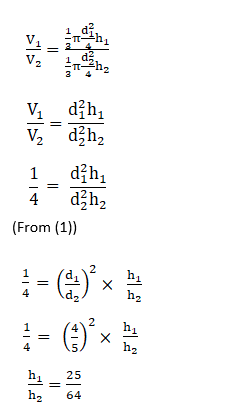(Using equation (2))

Therefore, Ratio of height of two cones is 25:64.

Question 6: The slant height of a conical mountain is 2.5 km and the area of its base is 1.54 km2. Find the height of the mountain.

Solution:

Given:

Slant height of conical mountain = 2.5 km

Area of its base = 1.54 km2

Let the radius of base be ‘r’ km, height of the mountain is ‘h’ km and slant height be ‘l’ km

Area of base = πr2

1.54 = πr2

1.54 = 22/7 r2

or r = 0.7 km

We know that, l2 = r2 + h2

(2.5)2 = (0.7)2 + h2

6.25 – 0.49 = h2

or h = 2.4 km

Question 7: The sum of the radius of the base and the height of a solid cylinder is 37 metres. If the total surface area of the cylinder be 1628 sq metres, find its volume.

Solution:

Height = h

The sum of the radius of the base and the height of a solid cylinder is 37 metres.

⇨ r + h = 37 m …(1)

Total surface area of the cylinder = 2πr(r + h)

r = 7

From (1): 7 + h = 37

h = 30

Again,

Volume of the cylinder = πr2 h

Question 8: The surface area of a sphere is 2464 cm2. If its radius be doubled, then what will be the surface area of the new sphere?

Solution:

Surface area of a sphere = 2464 cm2 (Given)

4π r2 = 2464

Now,

Surface area of a sphere with radius 2r = 4π (2r)2

= 4 x 4π r2

= 4 x 2464

= 9856 cm2

Question 9: A military tent of height 8.25 m is in the form of a right circular cylinder of base diameter 30 m and height 5.5 m surmounted by a right circular cone of same base radius. Find the length of canvas used in making the tent, if the breadth of the canvas is 1.5 m.

Solution:

A military tent is made as a combination of right circular cylinder and right circular cone on top.

Given :

Total Height of tent = h = 8.25 m

Base diameter of tent = 30 m, then

Base radius of tent = r = 30/2 m = 15 m

Height of right circular cylinder = 5.5 m

Base radius of cone = 15 m

Let slant height of cone = l m

Now,

Curved surface area of right circular cylindrical part of tent = 2πrh

and

Height of conical part = total height of tent – height of cylindrical part

Height of cone = 8.25 – 5.5 = 2.75 m

l2 = h2 + r2

= 2.752 + 152

= 232.5625

or l = 15.25 m

Curved surface area of conical part of the tent = πrl

Total surface area of the tent = Curved surface area of cylindrical part + curved surface area of conical part

Total surface area of tent = 2πrh + πrl

= πr(2h + l)

= 22/7 × 15 × (2 × 5

= 1237.5

Total surface area of tent is 1237.5 m2

Breadth of canvas used = 1.5 m

Length of canvas used x breadth of canvas used = Total surface area of tent

Length of canvas used = 1237.5\1.5 = 825

Length of canvas used is 825 m.

Question 10: A tent is in the shape of a right circular cylinder up to a height of 3 m and conical above it. The total height of the tent is 13.5 m and the radius of its base is 14 m. Find the cost of cloth required to make the tent at the rate of Rs.80 per square metre. [take π = 22/7]

Solution:

The tent is made as a combination of right circular cylinder and right circular cone on top.

Height of cylindrical part of the tent = h = 3 m

Radius of its base = r = 14 m

Total height of the tent = 13.5 m

Curved surface area of cylindrical part of tent = 2πrh

= 2 × 22/7 × 14 × 3

= 264 m2

Height of conical part of the tent = total height of tent – height of cylindrical part

Height of conical part of the tent = 13.5 – 3 m =10.5 m

Let the slant height of the conical part be l.

l2 = h2 + r2 (used cone height)

= 110.25 + 196

= 306.25

or l = 17.5 m

Curved surface area of conical part of tent = πrl

= 22/7 × 14 × 17.5

= 770

Total surface area of tent = Curved surface area of cylindrical part of tent + Curved surface area of conical part of tent.

Total Surface area of tent = 264 + 770

= 1034 m2

Cloth required = Total Surface area of tent = 1034 m2

Cost of cloth = Rs 80/m2 (given)

Total cost of cloth required = Total surface area of tent × Cost of cloth

= 1034 × Rs. 80

= Rs. 82720

Cost of cloth required to make the tent is Rs. 82720

## Exercise 17B

Question 1: A solid metallic cuboid of dimensions 9m× 8m×2m is melted and recast into solid cubes of edge 2m. Find the number of cubes so formed.

Solution:

A solid metallic cuboid of dimensions 9m× 8m×2m (given)

Length (l) = 9 m

Height (h) = 2 m

Edge of a cube (a) = 2 m

Let n be the number of required cubes.

To find: Value of n

Number of cubes = (volume of a cuboid)/ volume of each cube)

= lbh/a3

= (9x8x2)/(2x2x2)

= 18

Therefore, total number of 18 cubes required.

Question 2: A cone of height 20 cm and radius of base 5 cm is made up of modelling clay. A child reshapes it in the form of a sphere. Find the diameter of the sphere.

Solution:

Radius of the cone = r = 5cm and

Height of the cone = h = 20cm

Let the radius of the sphere = R

As per given statement,

Volume of sphere = Volume of cone

4/3 πR3 = 1/3πr2h

4R3 = 5 × 5 × 20

R = 5 cm

Diameter of the sphere = 2R = 2 x 5 = 10 cm

Question 3: Metallic spheres of radii 6 cm, 8 cm and 10 cm respectively are melted to form a single solid sphere. Find the radius of the resulting sphere.

Solution:

Radius of 1st sphere = r1 = 6 cm

Radius of 2nd sphere =r2 = 8 cm and

Radius f third sphere = r3 = 10 cm

Let radius of the resulting sphere = R

Now,

Volume of resulting sphere = Volume of three metallic spheres

4/3 πR3 = 4/3π (r13 + r23 + r33)

= 4/3π (63 + 83 + 103)

R3 = 1728

or R = 12 cm

Radius of the resulting sphere is 12cm.

Question 4: A solid metal cone with base radius of 12 cm and height 24 cm is melted to form solid spherical balls of diameter 6 cm each. Find the number of balls thus formed.

Solution:

Let the number of balls formed are n

As per statement,

Volume of metal cone = Total volume of n spherical balls

Volume of cone = n(Volume of any spherical ball)

1/3 π r2 h = n (4/3 π r3)

122 x 24 = n x 108

or n = 32

Therefore, 32 spherical balls can be formed.

Question 5: The radii of internal and external surfaces of a hollow spherical shell are 3 cm and 5 cm respectively. It is melted and recast into a solid cylinder of diameter 14 cm. Find the height of the cylinder.

Solution:

Let r1 and r2 be the internal and external base radii of spherical shell.

r1 = 3 cm, and r2 = 5 cm

Base radius of solid cylinder, r = 7 cm

Let the height of the cylinder = h

As per given statement:

The hollow spherical shell is melted into a solid cylinder, so

Volume of solid cylinder = Volume of spherical shell

πr2h = 4/3 π(r23 – r13)

⇨ 49h = 4/3(125 – 27)

or h = 8/3 cm

Question 6: The internal and external diameters of a hollow hemispherical shell are 6 cm and 10 cm, respectively. It is melted and recast into a solid cone of base diameter 14 cm. Find the height of the cone so formed.

Solution:

Internal radius of hemisphere = 3 cm and external radius of hemisphere = 5 cm

Diameter of cone = 14 cm

Radius of cone = 7 cm

Now,

Volume of the hollow hemisphere = Volume of the cone

Volume of cone = 1/3 π r2 h

Volume of the hollow hemisphere = 2/3 π(R3 – r3)

2/3 π(R3 – r3) = 1/3 π r2 h

2/3 x 22/7 (53 – 33) = 1/3 x 22/7 x 72 x h

2 x 98 = 49h

or h = 4 cm

Height of the cone formed is 4 cm.

Question 7: A copper rod of diameter 2 cm and length 10 cm is drawn into a wire of uniform thickness and length 10 m. Find the thickness of the wire.

Solution:

Recall MU: 1m = 1000 mm and 1 cm = 10 mm

Diameter of the copper Rod = 2cm

Radius of the copper rod = r= 1 cm

Length of the Rod = h = 10 cm

Length of the wire = h = 10 m = 1000cm

Let suppose the radius of the wire = R

From the statement: Volume of the rod = volume of the wire

πr2h = πR2H

1 × 1 × 10 = 1000 × R

or R = 1/10 cm or 01. cm

Diameter of the wire = 2r = 2 × 0.1 = 0.2 cm

Therefore, the thickness of the wire is 0.2 cm or 2mm.

Question 8: A hemispherical bowl of internal diameter 30 cm contains some liquid. This liquid is to be poured into cylindrical bottles of diameter 5 cm and height 6 cm each. Find the number of bottles necessary to empty the bowl.

Solution:

Internal diameter of hemispherical bowl = 30 cm

Volume = 2/3 πr3

= 2/3 π (15)3

= 2250 π cm3

Diameter of the cylindrical bottle = 5 cm

Volume of one cylindrical bottle = πr2h

= π x (2.5)2 x 6

= 37.5π cm3

Now,

Amount of water in n bottles = Amount of water in bowl

n × 37.5π = 2,250π

or n = 60

So, 60 numbers of bottles are required to empty the bowl.

Question 9: A solid metallic sphere of diameter 21 cm is melted and recast into a number of smaller cones, each of diameter 3.5 cm and height 3 cm. Find the number of cones so formed.

Solution:

Diameter of sphere = 21 cm

Height of the cone = 3 cm

Radius of the cone = 7/4 cm

Volume of the sphere = 4/3 πr3 = 4/3 x π x (21/2)3 cm3

Volume of cone = 1/3 πr2 h = 1/3 π (7/4)2 x 3 cm3

Let n be the number of cone formed, then

n = (Volume of the sphere) / (Volume of cone)

n = (4/3 x π x (21/2)3) / (1/3 π (7/4)2 x 3)

= 504

Number of cones formed = 504

Question 10: A spherical cannon ball, 28 cm in diameter is melted and recast into a right circular conical mould, base of which is 35 cm in diameter. Find the height of the cone.

Solution:

Radius of cannon ball = 14 cm

Volume of cannon ball = 4/3 π r3 = 4/3 π (14)3

Radius of Cone = 35/2 cm

Volume of Cone = 1/3 πr2 h = 1/3 π(35/2)2 h

Let h be the height of the cone.

From statement:

Volume of cannon ball = Volume of Cone

⇨ 4/3 π (14)3 = 1/3 π(35/2)2 h

h = 35.84

Therefore, height of the cone is 35.84 cm.

## Exercise 17C

Question 1: A drinking glass is in the shape of a frustum of a cone of height 14 cm. The diameters of its two circular ends are 16 cm and 12 cm. Find the capacity of the glass.

Solution:

Diameter of lower circular end of glass = 12 cm

Radius of lower circular end = r = 12/2 = 6 cm

Diameter of upper circular end of glass = 16 cm

Radius of lower circular end = R = 16/2 = 8 cm

Height of glass = h = 14 cm

Capacity of glass = 1/3 πh(R2 + r2 + Rr)

= 1/3 x 22/7 x 14(82 + 62 + 8×6)

= 44/3(64 + 36+48)

= 2170.67

Capacity of glass is 2170.67 cm3

Question 2: The radii of the circular ends of a solid frustum of a cone are 18 cm and 12 cm and its height is 8 cm. Find its total surface area. [Use π= 3.14]

Solution:

Height of frustum = h = 8 cm

Radius of lower circular end = r = 12 cm

Radius of upper circular end = R = 18 cm

Let l = slant height

Slant height = l2 = (R-r)2 + h2 cm

= 36 + 64

= 100

or l = 10 cm

We know, Total surface area of frustum = πr2 + πR2 + π(R + r)l cm2

= π × 122 + π × 18^ + π × (18 + 12) × 10 cm2

= 3.14(144 + 324 + 300)

= 2411.52

Total surface area of frustum is 2411.52 cm2

Question 3: A metallic bucket, open at the top, of height 24 cm is in the form of the frustum of a cone, the radii of whose lower and upper circular ends are 7 cm and 14 cm, respectively. Find

(i) the volume of water which can completely fill the bucket;

(ii) the area of the metal sheet used to make the bucket.

Solution:

Radius of lower circular end = r = 7 cm

Radius of upper circular end = R = 14 cm

Height of bucket = h = 24 cm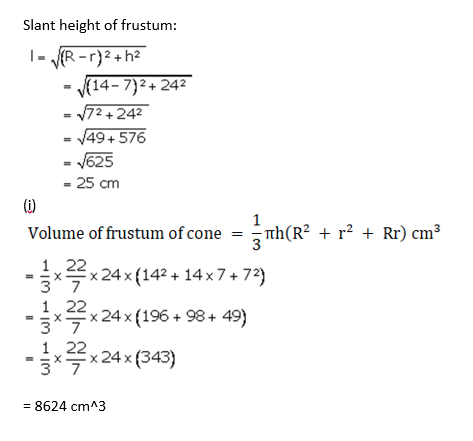(ii)Curved surface area = πl( R+r)

= 22/7 x 25 x (14+7)

= 1650 cm2

Area of the base of bucket = πr2

(consider lower base of bucket)

= 22/7 x 7 x 7

= 154 cm2

Area of metal sheet used to make the bucket = curved surface area + Area of the base

= 1650 + 154

= 1804

Area of metal sheet used to make the bucket is 1804 cm2

Question 4: A container, open at the top, is in the form of a frustum of a cone of height 24 cm with radii of its lower and upper circular ends as 8 cm and 20 cm, respectively. Find the cost of milk which can completely fill the container at the rate of Rs. 21 per litre.

Solution:

Radius of lower circular end = r = 8 cm

Radius of upper circular end = R = 20 cm

Cost of 1 litre milk = Rs. 24

Height of frustum container = h = 24 cm

Volume of frustum of cone = 1/3π h(R2 + r2 + Rr) cm3

Volume of milk completely fill the container = Volume of frustum of cone

= 1/3 π x 24(202 + 82 + 20×8)

= 15689.14 cm3

= 15.68914 litres

Since 1 litre is 1000 cm3

Cost of 1 liter milk = Rs. 21

Cost of 15.68914 liter milk = Rs. 21 x 15.68914 = Rs. 329.47

Question 5: A container made of a metal sheet open at the top is of the form of a frustum of cone, whose height is 16 cm and the radii of its lower and upper circular edges are 8 cm and 20 cm respectively. Find

(i)the cost of metal sheet used to make the container if it costs Rs. 10 per 100 cm2.

(ii) the cost of milk at the rate of Rs. 35 per liter which can fill it completely.

Solution:

Radius of lower end = r = 8 cm

Radius of upper end = R = 20 cm

Height of container frustum = h = 16 cm

Cost of 100 cm2 metal sheet = Rs 10

So, Cost of 1 cm2 metal sheet = 10/100 = Rs 0.1

Let l be the slant height.

l2 = (R-r)2 + h2

= (20-8)2 + 162

= 256 + 144

= 400

or l= 20cm

Surface area of frustum of the cone = πr2 + π(R + r)l cm2

= π[ (20 + 8 )20 + 82]

= π[560 + 64]

= 624 X ( 22/7 )

= 1961.14 cm2

(i) Find cost of metal sheet used to make the container if it costs Rs. 10 per 100 cm2

Cost of metal sheet per 100 cm2 = Rs. 10

Cost of metal for Rs. 1961.14 cm2 = (1961.14 x10)/100 = Rs. 196.114

(ii) Find the volume of frustum:

Volume of frustum = 1/3 πh(r2 + R2 + rR)

= 1/3 x 22/7 x 16(82 + 202 + 8×20)

= 1/3 x 22/7 x 16(64 + 400 + 160)

= 10345.4208 cm3

= 10.345 liters

Using

1000cm3 = 1 litre

1 cm3 = 1/1000 litre

Cost of 1 liter milk = Rs. 35

Cost of 10.345 liter milk = Rs. 35 x 10.345 = Rs. 363 (approx)

Question 6: The radii of the circular ends of a solid frustum of a cone are 33 cm and 27 cm, and its slant height is 10 cm. Find its capacity and total surface area. [take π = 22/7]

Solution: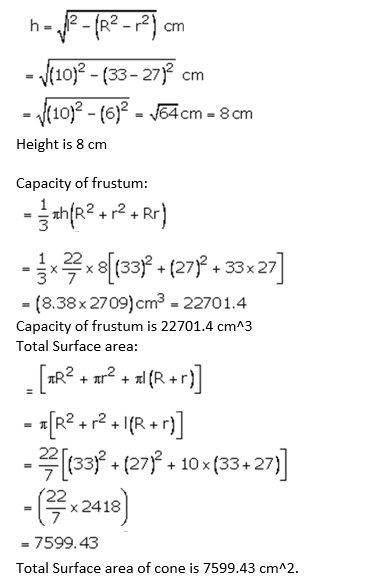Question 7.

A bucket is in the form of a frustum of a cone. Its depth is 15 cm and the diameters of the top and the bottom are 56 cm and 42 cm respectively. Find how many litres of water the bucket can hold. [take π = 22/7]

Solution:

Depth of the bucket = height of frustum = h = 15 cm

Diameter of top of bucket = 56 cm

Radius of top = R = 56/2 = 28 cm

Diameter of bottom of bucket = 42 cm

Radius of bottom = r = 42/2 = 21 cm

Volume of frustum of cone = 1/3π h(R2 + r2 + Rr) cm3

= 1/3 x 22/7 x 15(282 + 212 + 28×21)

= 22 x 5 x 259

= 28490

Since Volume of water bucket can hold = volume of bucket which is in form of frustum

So, volume of water bucket can hold is 28490 cm3 or = 28.49 litres

Question 8: A bucket made up of a metal sheet is in the form of a frustum of a cone of height 16 cm and radii of its lower and upper ends are 8 cm and 20 cm respectively. Find the cost of the bucket if the cost of metal sheet used is Rs. 15 per 100 cm2. [use π = 3.14]

Solution:

Radius of lower circular end = r = 8 cm

Radius of upper circular end = R = 20 cm

Height of container frustum = h = 16 cm

Cost of 100 cm2 metal sheet = rs. 15

Cost of 1 cm2 metal sheet = 15/100 = Rs. 0.15

Slant height, l2 = (R-r)2 + h2

= (20-8)2 + 162

= 144 + 256

= 400

or l = 20 cm

Now,

Area of metal sheet used = (Total surface area of frustum)- (Area of upper circle) …(1)

Area of upper circle = πR2

Total surface area of frustum = πr2 + πR2 + π(R + r)l cm2

(1)⇨

Area of metal sheet used = (πr2 + πR2 + π(R + r)l) – πR2

= πr2 + π(R + r)l

= π(82 + (20 + 8)20)

= 1959.36 cm3

Again,

Cost of 1959.36 cm2 metal sheet = 1959.36 × cost of 1 cm2 metal sheet

= 1959.36 × Rs. 0.15

= Rs.293.904

Question 9: A bucket made up of a metal sheet is in the form of frustum of a cone. Its depth is 24 cm and the diameters of the top and bottom are 30 cm and 10 cm respectively. Find the cost of milk which can completely fill the bucket at the rate of Rs. 20 per litre and the cost of metal sheet used if it costs Rs. 10 per 100 cm2. [use π = 3.14]

Solution:

Let r = 5 cm, R = 15 cm and h = 24 cm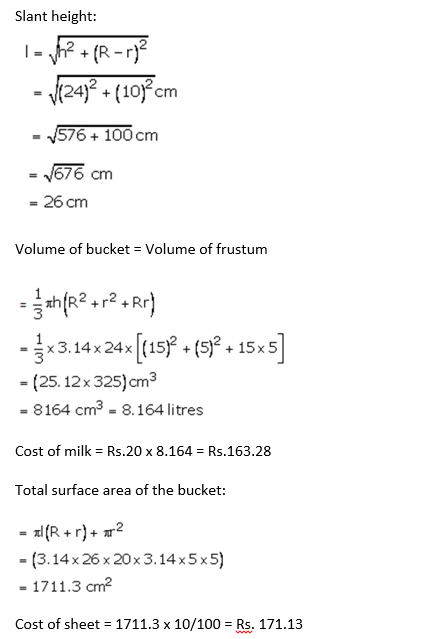Question 10: A container in the shape of a frustum of a cone having diameters of its two circular faces as 35 cm and 30 cm and vertical height 14 cm, is completely filled with oil. If each cm3 of oil has mass 1.2 g, then find the cost of oil in the container if it costs Rs. 40 per kg.

Solution:

Diameter of top of container = 35 cm

Radius of top = R = 35/2 = 17.5 cm

Diameter of bottom of container = 30 cm

Radius of bottom = r = 30/2 = 15 cm

1 cm3 of oil = 1.2g of oil, so, Cost of 1 kg oil = Rs. 40

Height of frustum = h = 14 cm

Volume of frustum of cone = 1/3π h(R2 + r2 + Rr) cm3

Volume of oil in container = 1/3 x 22/7 x 14(17.52 + 152 + 17.5 x 15)

= 22/3 × 2 × 793.75

=34925/3

Volume of oil in container = 11641.667 cm3 or 11641.667 × 1.2 g = 13970.0004 g or 13.970 kg

(As 1000 g = 1 kg)

Cost of 13.970 kg oil = Rs. 20 x 13.970 = Rs. 558.

## Exercise 17D

Question 1: A river 1.5 m deep and 36 m wide is flowing at the rate of 3.5 km/hr. Find the amount of water (in cubic metres) that runs into the sea per minute.

Solution:

Depth of the river = 1.5 m

Width of the river = 36 m

Using units:

1 hour = 60 minutes

1 km = 1000 m

Flow rate of river = 3.5 km/hr

= (3.5) x 1000)/1×60) m/min

= 350/6 m/min

The amount of water that runs into sea per minute =

350/6 × 1.5 × 36 = 350 × 1.5 × 6 = 3150

The amount of water that runs into sea per minute is 3150 m3/min.

Question 2: The volume of a cube is 729 cm3. Find its surface area.

Solution:

Volume of a cube is 729 cm3 (given)

we know, Volume of the cube = (side)3

(side)3 = 729

or Side = 9

Each side measure of a cube is 9 cm.

Now,

Total surface area of the cube = 6(side)2

= 6 × 92

= 6 × 81

= 486

Total surface area of the cube is 486 cm2.

Question 3: How many cubes of 10 cm edge can be put in a cubical box of 1 m edge?

Solution:

Let ‘a’ be edge if a cube, so edge = 1m or 100 cm

Volume of cube of 100 cm edge = (side)3

= (100)3

= 1000000 cm3

Volume of cubes of 10cm edge = 103 = 1000 cm3

Now,

Number of required cubes = (Volume of cube of 100 cm edge)/(Volume of cubes of 10cm edge)

= 1000000/1000000

= 1000

Question 4: Three cubes of iron whose edges are 6 cm, 8 cm and 10 cm, respectively are melted and formed into a single cube. Find the edge of the new cube formed.

Solution:

Edge of the first cube = 6 cm

Volume of the first cube = (side)3 = (6)3 cm3

Edge of the second cube = 8 cm

Volume of the second cube = (side)3 = (8)3 cm3

Edge of the third cube = 10 cm

Volume of the third cube = (side)3 = (10)3 cm3

Let “a” be side edge of the new formed cube.

Volume of the formed cube = Volume of the First cube + Volume of the Second cube + Volume of the Third Cube

a3 = 63 + 83 + 103

= 216 + 512 + 1000

= 1728

0r a = 12

Edge of the new cube is 12 cm.

Question 5: Five identical cubes, each of edge 5 cm, are placed adjacent to each other. Find the volume of the resulting cuboid.

Solution:

Edge of the given Cube = 5 cm

When 5 identical cubes are placed adjacent to each other, the length of cuboid formed is 5 x 5 = 25 cm

Now, Volume of the resulting cuboid = lbh

= 25 x 5 x 5

= 625 cm3

Question 6: The volumes of two cubes are in the ratio 8 : 27. Find the ratio of their surface areas.

Solution:

Ratio of two cube = 8:27

Let volumes of the two cubes be 8x and 27x

Let a and b be the sides of first cube and second cube respectively.

Volume of cube = (side)3

8x = a3

and 27x = b3

Formula to find surface area = 6(side)2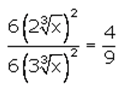The required ratio is 4:9.

Question 7: The volume of a right circular cylinder with its height equal to the radius is 25 1/7 cm3. Find the height of the cylinder.

Solution:

Volume of the right circular Cylinder = 176/7 cm3

Height of the right circular cylinder = Radius of the right circular cylinder

⇨ h = r

We know, Volume of the right circular Cylinder = πr2h

πr2h = 176/7

22/7 × h2 × h = 176/7

h3 = 8

or h = 2 cm

Height of the cylinder is 2 cm.

Question 8: The ratio between the radius of the base and the height of a cylinder is 2 : 3. If the volume of the cylinder is 12936 cm3, then find the radius of the base of the cylinder.

Solution:

Volume of the cylinder = 12936 cm3

Ratio of the base and the height of a cylinder is 2:3

Let 2x be the radius and 3x be the height.

Volume of the cylinder = 12936 cm3

πr2h = 12936

22/7 x (2x)2(3x) = 12936

x3 = 343

or x = 7

Radius of the base is 14 cm.

Question 9: The radii of two cylinders are in the ratio of 2 : 3 and their heights are in the ratio of 5 : 3. Find the ratio of their volumes.

Solution:

The radii of two cylinders are in the ratio of 2 : 3 and their heights are in the ratio of 5 : 3.

Let 2r and 3r are the radii and 5h and 3h be the heights.

= 20/27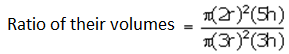Ratio of their volumes is 20:27

Question 10: 66 cubic cm of silver is drawn into a wire 1 mm in diameter. Calculate the length of the wire in metres.

Solution:

Diameter of the wire = 1 mm

Radius of wire = r = 1/2 mm = 0.5 mm = 0.05 cm

Let the length of the wire be h

Volume of the wire = 66 cm3

πr2h = 66

22/7 x 0.05 × 0.05 × h = 66

h = (66x7x400)/22

h = 8400

Length of the wire is 8400 cm or 84 m.

## R S Aggarwal Solutions for Class 10 Maths Chapter 17 Volume and Surface Area of Solids

In this chapter students will study important concepts on Volume and Surface Area of Solids as listed below:

• Volume and Surface Area of Solids Introduction

Solids can be defined as objects having definite shape and size.

• Volume and Surface Area of Cuboid
• Volume and Surface Area of Cube
• Volume and Surface Area of the cylinder and hollow cylinder
• Volume and Surface Area of cone
• Volume and Surface Area of sphere and hemisphere
• Volume and Surface Area of a combination of solids
• Volume and Surface Area of a frustum of a cone
• Conversion of solid from one shape to another and mixed problems

### Key Features of RS Aggarwal Solutions for Class 10 Maths Chapter 17 Volume and Surface Area of Solids

1. R S Aggarwal solutions is a set of problems based on Volume and surface area of solid figures – sphere, cube, rectangular solid with and without top, cylinder, and cone.

2. The combination of diagrams and corresponding formulas will help students gain a thorough understanding of these concepts.

3. Easy for quick revision.

4. Helps students to solve complex problems at their own pace.